Atmos. Chem. Phys., 20, 6651–6670, 2020
https://doi.org/10.5194/acp-20-6651-2020
Atmos. Chem. Phys., 20, 6651–6670, 2020
https://doi.org/10.5194/acp-20-6651-2020

Research article 05 Jun 2020

Research article | 05 Jun 2020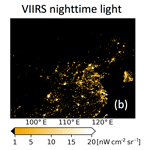# Inverse modeling of SO2 and NOx emissions over China using multisensor satellite data – Part 2: Downscaling techniques for air quality analysis and forecasts

Inverse modeling of SO2 and NOx emissions over China using multisensor satellite data – Part 2: Downscaling techniques for air quality analysis and forecasts
Yi Wang1, Jun Wang1,2, Meng Zhou1, Daven K. Henze3, Cui Ge2,4, and Wei Wang5 Yi Wang et al.
• 1Interdisciplinary Graduate Program in Informatics, The University of Iowa, Iowa City, IA 52242, USA
• 2Department of Chemical and Biochemical Engineering, and Center for Global and Regional Environmental Research, The University of Iowa, Iowa City, IA 52242, USA
• 3Department of Mechanical Engineering, University of Colorado, Boulder, CO 80309, USA
• 4South Coast Air Quality Management District, Diamond Bar, CA 91765, USA
• 5China National Environmental Monitoring Center, Beijing 100012, China

Correspondence: Jun Wang (jun-wang-1@uiowa.edu) and Yi Wang (yi-wang-4@uiowa.edu)

Abstract

Top-down emission estimates provide valuable up-to-date information on pollution sources; however, the computational effort and spatial resolution of satellite products involved with developing these emissions often require them to be estimated at resolutions that are much coarser than is necessary for regional air quality forecasting. This work thus introduces several approaches to downscaling coarse-resolution ($\mathrm{2}{}^{\circ }×\mathrm{2.5}{}^{\circ }$) posterior SO2 and NOx emissions for improving air quality assessment and forecasts over China in October 2013. As in Part 1 of this study, these $\mathrm{2}{}^{\circ }×\mathrm{2.5}{}^{\circ }$ posterior SO2 and NOx emission inventories are obtained from GEOS-Chem adjoint modeling with the constraints of OMPS SO2 and NO2 products retrieved at 50 km×50 km at nadir and $\sim \mathrm{190}\phantom{\rule{0.125em}{0ex}}\mathrm{km}×\mathrm{50}\phantom{\rule{0.125em}{0ex}}\mathrm{km}$ at the edge of ground track. The prior emission inventory (MIX) and the posterior GEOS-Chem simulations of surface SO2 and NO2 concentrations at coarse resolution underestimate observed hot spots, which is called the coarse-grid smearing (CGS) effect. To mitigate the CGS effect, four methods are developed: (a) downscale $\mathrm{2}{}^{\circ }×\mathrm{2.5}{}^{\circ }$ GEOS-Chem surface SO2 and NO2 concentrations to the resolution of $\mathrm{0.25}{}^{\circ }×\mathrm{0.3125}{}^{\circ }$ through a dynamic downscaling concentration (MIX-DDC) approach, which assumes that the $\mathrm{0.25}{}^{\circ }×\mathrm{0.3125}{}^{\circ }$ simulation using the prior MIX emissions has the correct spatial distribution of SO2 and NO2 concentrations but a systematic bias; (b) downscale surface NO2 simulations at $\mathrm{2}{}^{\circ }×\mathrm{2.5}{}^{\circ }$ to $\mathrm{0.05}{}^{\circ }×\mathrm{0.05}{}^{\circ }$ according to the spatial distribution of Visible Infrared Imaging Radiometer Suite (VIIRS) nighttime light (NL) observations (e.g., NL-DC approach) based on correlation between VIIRS NL intensity with TROPOspheric Monitoring Instrument (TROPOMI) NO2 observations; (c) downscale posterior emissions (DE) of SO2 and NOx to $\mathrm{0.25}{}^{\circ }×\mathrm{0.3125}{}^{\circ }$ with the assumption that the prior fine-resolution MIX inventory has the correct spatial distribution (e.g., MIX-DE approach); and (d) downscale posterior NOx emissions using VIIRS NL observations (e.g., NL-DE approach). Numerical experiments reveal that (a) using the MIX-DDC approach, posterior SO2 and NO2 simulations improve on the corresponding MIX prior simulations with normalized centered root mean square error (NCRMSE) decreases of 63.7 % and 30.2 %, respectively; (b) the posterior NO2 simulation has an NCRMSE that is 17.9 % smaller than the prior when they are both downscaled through NL-DC, and NL-DC is able to better mitigate the CGS effect than MIX-DDC; (c) the simulation at $\mathrm{0.25}{}^{\circ }×\mathrm{0.3125}{}^{\circ }$ using the MIX-DE approach has NCRMSEs that are 58.8 % and 14.7 % smaller than the prior $\mathrm{0.25}{}^{\circ }×\mathrm{0.3125}{}^{\circ }$ MIX simulation for surface SO2 and NO2 concentrations, respectively, but the RMSE from the MIX-DE posterior simulation is slightly larger than that from the MIX-DDC posterior simulation for both SO2 and NO2; (d) the NL-DE posterior NO2 simulation also improves on the prior MIX simulation at $\mathrm{0.25}{}^{\circ }×\mathrm{0.3125}{}^{\circ }$, but it is worse than the MIX-DE posterior simulation; (e) in terms of evaluating the downscaled SO2 and NO2 simulations simultaneously, using the posterior SO2 and NOx emissions from joint inverse modeling of both species is better than only using one (SO2 or NOx) emission from corresponding single-species inverse modeling and is similar to using the posterior emissions of SO2 and NOx emission inventories respectively from single-species inverse modeling.

Forecasts of surface concentrations for November 2013 using the posterior emissions obtained by applying the posterior MIX-DE emissions for October 2013 with the monthly variation information derived from the prior MIX emission inventory show that (a) the improvements of forecasting surface SO2 concentrations through MIX-DE and MIX-DDC are comparable; (b) for the NO2 forecast, MIX-DE shows larger improvement than NL-DE and MIX-DDC; (c) NL-DC is able to better decrease the CGS effect than MIX-DE but shows larger NCRMSE; (d) the forecast of surface O3 concentrations is improved by MIX-DE downscaled posterior NOx emissions. Overall, for practical forecasting of air quality, it is recommended to use satellite-based observation already available from the last month to jointly constrain SO2 and NO2 emissions at coarser resolution and then downscale these posterior emissions at finer spatial resolution suitable for regional air quality modeling for the present month.

Share
1 Introduction

Simulations and forecasts of surface concentrations of SO2 and NO2, the two criteria pollutants in the atmosphere defined by the US EPA and China, are important for studying their impacts on air quality and public health (Ghozikali et al., 2016). Their accuracy depends not only on reliability of meteorological fields and correct representation of chemical processes in the air quality model (Gao et al., 2016; Ge et al., 2017) but also on the fidelity of the emissions used in the latter. For the same region and time, different emission inventories can lead to differences of up to 100 % for surface SO2 and NO2 simulations (Y. Wang et al., 2016). Additionally, model resolution also plays an important role (Kharol et al., 2017), as the simulated concentration of these short-lived species only represents the average of a grid cell in which the high concentrations of SO2 and NO2 from source regions and (or) strong spatial variation of these species are smeared out. This is called the coarse-grid smearing (CGS) effect, and it depends on the species lifetimes, the spatial distribution of emissions, and the model (and inventory) resolution. The lifetime for SO2 in the lower troposphere is less than 1 d in the summer and 1 or 2 d in winter (Lee et al., 2011), and it is several hours for NO2 (Lin et al., 2010); their smearing length scales (Palmer et al., 2003) are on the order of 100 km (Lee et al., 2011; Martin et al., 2003). Xing et al. (2015) showed that surface SO2 and NO2 concentrations from the Weather Research and Forecasting Model (WRF)–Community Multiscale Air Quality (CMAQ) model simulations at 108 km×108 km resolution were underestimated when evaluated with urban network observations and overestimated relative to rural networks.

Obtaining accurate and timely emission estimates can be challenging. The bottom-up approach, which integrates activity data and emission factors, is widely used to generate inventories (Li et al., 2017a; Janssens-Maenhout et al., 2015; Kurokawa et al., 2013). These bottom-up emissions have uncertainties larger than 30 % at the regional scale for both SO2 and NOx over China (Kurokawa et al., 2013; Li et al., 2017a). When used to simulate air quality with a chemical transport model (CTM), these emission estimates are gridded to regular grid cells (of  1 or finer) through locations of major manufacturing facilities and power plants and proxy data such as population distributions and road networks (Zheng et al., 2017; Streets et al., 2003). Consequently, uncertainties of emission estimates at the grid-cell scale are larger than at the country scale. Moreover, bottom-up inventories usually have a time lag of at least 1 year, as it takes time to collect all the data required to generate them (Liu et al., 2018). Outdated emission inventories increase the uncertainty of simulations and forecasts, especially for China where emissions change quickly due to rapid economic development and implementation of emission control policies (Zheng et al., 2018; Y. Wang et al., 2016).

Over the past two decades, many satellites have provided vertical column density (VCD) data of SO2 and NO2 and aerosol optical depth (AOD) retrievals globally; these data have been used to constrain emission estimates with the following approaches at various spatial resolutions. The mass balance approach (Lee et al., 2011; Martin et al., 2003; Koukouli et al., 2018) and the finite-difference mass balance method (Lamsal et al., 2011) were developed to use VCD retrievals of SO2 and NO2 from the Global Ozone Monitoring Experiment (GOME), GOME-2, SCanning Imaging Absorption spectroMeter for Atmospheric CHartographY (SCIAMACHY), Ozone Monitoring Instrument (OMI), and Ozone Mapper and Profiler Suite (OMPS) to constrain SO2 and NOx emissions at spatial resolutions in the range of 25 to 250 km. The accuracy, however, decreases as spatial resolution becomes finer (Turner et al., 2012), because the transport is not explicitly accounted for in these approaches. The emission strength of SO2 point sources that are larger than 30 kt yr−1 can be estimated through a linear regression between OMI VCDs and emission strength (Fioletov et al., 2016), and the approach was used to build a global SO2 emission inventory at $\mathrm{0.1}{}^{\circ }×\mathrm{0.1}{}^{\circ }$ (Liu et al., 2018). Advanced data assimilation approaches including the four-dimension variational data assimilation (4D-Var) (Qu et al., 2019a, b, 2017; Y. Wang et al., 2016a, 2020; Wang et al., 2012; Xu et al., 2013; Kurokawa et al., 2009) and the ensemble Kalman filter (EnKF) approach (Miyazaki et al., 2012, 2017) were developed to use satellite SO2 and NO2 columns densities and AOD retrievals to constrain emissions at low spatial resolutions (> 50 km) as these approaches are computationally intensive. Some variations of the 4D-Var and Kalman filter approaches were developed to save computational time at the expense of accuracy or temporal resolution (Qu et al., 2017; Kong et al., 2019; Mijling and van der A, 2012; Ding et al., 2015).

The mismatch among the resolutions of emission inventories, CTMs, and satellite observations has prompted previous development of downscaling methods. For example, the popular OMI has a footprint size of 13 km×24 km at nadir and 26 km×128 km at the swath edge that is too coarse to capture the urban NO2 plume without oversampling. Consequently, a spatial weighting kernel derived from the CMAQ simulation at finer spatial resolution was developed to downscale OMI NO2 retrievals to 1.33 km×1.33 km (Kim et al., 2018, 2016; Goldberg et al., 2017). The resulting high-spatial-resolution OMI NO2 data was further applied to constrain emissions, which showed an underestimate in the bottom-up NOx inventories in Seoul, South Korea, during the Korea–United States Air Quality Study (KORUS-AQ) (Goldberg et al., 2019). In cases where model grid cells are larger than satellite footprints, Lamsal et al. (2008) applied the ratio between the local OMI NO2 column to mean OMI field over a $\mathrm{2}{}^{\circ }×\mathrm{2.5}{}^{\circ }$ GEOS-Chem grid cell to derive local surface-VCD scaling factors, which were used to infer improved surface NO2 concentrations. An inverse distance weighting technique was applied to interpolate emissions and initial and boundary species conditions from coarse resolution to fine resolution for nested CTM simulations (Yahya et al., 2017, 2016; Hong et al., 2017), but it was not able to capture hot spots in the downscaled fields.

The CGS effect, combined with the sharp spatial variations of surface SO2 and NO2 concentrations, introduces challenges when comparing model simulations with in situ observations. Y. Wang et al. (2016) showed the improvement when using posterior SO2 emissions constrained by OMI SO2 to simulate surface SO2 concentrations at a resolution of $\mathrm{2}{}^{\circ }×\mathrm{2.5}{}^{\circ }$ . However, this was illustrated for a rural site that is  100 km away from Beijing's urban center, and there are no strong SO2 sources around it, which means the CGS effect is minimal at this site. Kharol et al. (2015, 2017) found that surface SO2 and NO2 concentrations derived through scaling OMI SO2 and NO2 VCDs with vertical profiles from a CTM at a resolution of $\mathrm{0.1}{}^{\circ }×\mathrm{0.1}{}^{\circ }$ are a factor of 2 smaller than US EPA in situ observations. These underestimations are partly ascribed to the CGS effect, although uncertainty in vertical profiles also plays a role (Kharol et al., 2015, 2017; Bechle et al., 2013). They further showed that the underestimation decreases significantly when in situ observations are converted to represent the averages of larger areas through a linear regression function which is built by comparing simulations of SO2 between two spatial resolutions of 2.5 km×2.5 km and 30 km×30 km.

This paper, as the second of a two-part study, aims at using SO2 and NOx emissions constrained by OMPS SO2 and NO2 retrievals through 4D-Var (which is presented in Part 1, i.e., Wang et al., 2020) to improve air quality forecasts. Since the emission inventories in Part 1 are derived at the $\mathrm{2}{}^{\circ }×\mathrm{2.5}{}^{\circ }$ resolution to save computational resources and to account for the coarse spatial resolution of satellite data used (e.g., OMPS SO2 and NO2), the focus here is to develop novel methods to downscale coarse-resolution emission inventories or simulation results to generate fine-resolution surface SO2 and NO2 concentrations and evaluate them from an air quality forecasting point of view. High-resolution bottom-up emission inventories and Visible Infrared Imaging Radiometer Suite (VIIRS) nighttime lights contain geospatial information (such as roads, location of power plants, and residential areas) in fine spatial resolution for downscaling coarser-resolution anthropogenic emissions. Indeed, VIIRS nighttime light observations are shown to be good indicators of socioeconomic parameters including urbanization, economic activity, population (Bennett and Smith, 2017), and road density (Levin and Zhang, 2017) and have been used to map CO2 emissions (Ou et al., 2015) and derive surface PM2.5 concentrations at nighttime (J. Wang et al., 2016). Thus, it should also be promising to build relationships between VIIRS nighttime lights and both NO2 in the atmosphere and NOx emissions, which will be assessed here for their application in downscaling surface NO2 concentrations and NOx emissions.

We introduce data in Sect. 2. Section 3 presents the models for simulations and forecasts of surface SO2 and NO2, as well as the downscaling approaches. The improvements in the simulations and forecasts through various downscaling methods are provided in Sect. 4. Discussions of implications of the results and conclusions are followed in Sect. 5.

2 Data

## 2.1 In situ data of surface SO2, NO2, and O3

We obtained the in situ measurements of surface SO2, NO2, and O3 from the China National Environmental Monitoring Center for model evaluation. SO2 and NO2 are measured by various commercial instruments using the ultraviolet fluorescence method and the chemiluminescence method, respectively (Zhang and Cao, 2015). In the chemiluminescence method NO2 observations are obtained by measuring NO from decomposed NO2. This can result in a positive bias because NOz (all compounds that are products of the atmospheric oxidation of NOx) will also be reduced to NO. Steinbacher et al. (2007) showed that the ratio of NO2 to NOz (${r}_{{\mathrm{NO}}_{\mathrm{2}}}$) depends on the distance that NO2 plumes transport from the source. In other words, the longer the distance, the more the potential for oxidation of NO2 and hence the smaller ${r}_{{\mathrm{NO}}_{\mathrm{2}}}$; only 43 %–76 % and 70 %–83 % of real NO2 contribute to the measured value (NO2)m for rural and urban sites, respectively (Steinbacher et al., 2007). For this study, as observational sites are in cities, a maximum value of 0.83 is used to convert (NO2)m measurements to the NO2 concentrations, which are subsequently used for evaluating the model results. Additionally, we also test values for ${r}_{{\mathrm{NO}}_{\mathrm{2}}}$ in the range of 0.7 to 1.0.

## 2.2 VIIRS data for artificial light

The VIIRS on board the Suomi National Polar-orbiting Partnership (Suomi NPP) satellite was launched on 28 October 2011, and its day/night band (DNB) provides observations of nighttime lights with a spatial resolution of 750 m (Miller et al., 2013). Here, we use the VIIRS nighttime lights product that has excluded background noise as well as solar and lunar contamination and has screened out the data degraded by cloud cover and features unrelated to electric lighting (Elvidge et al., 2017a). This product is regridded to $\mathrm{0.05}{}^{\circ }×\mathrm{0.05}{}^{\circ }$ for October 2013 and to $\mathrm{0.05}{}^{\circ }×\mathrm{0.05}{}^{\circ }$ and $\mathrm{0.25}{}^{\circ }×\mathrm{0.25}{}^{\circ }$ for April 2018.

## 2.3 TROPOMI NO2 tropospheric VCD

The TROPOspheric Monitoring Instrument (TROPOMI) on board Sentinel-5 Precursor was launched on 13 October 2017, with a nadir footprint of 7 km×3.5 km, which is finer than that of all its predecessors. The TROPOMI NO2 tropospheric VCDs from the Royal Netherlands Meteorological Institute (KNMI) were retrieved using a differential optical absorption spectroscopy (DOAS) algorithm and validated with Pandora NO2 retrievals (Griffin et al., 2019). We grid the product to the $\mathrm{0.05}{}^{\circ }×\mathrm{0.05}{}^{\circ }$ resolution for April 2018 to investigate the relationship between VIIRS nighttime lights and NO2 tropospheric VCDs.

## 2.4 MIX emission inventory

MIX (Li et al., 2017a) is a mosaic of Asian anthropogenic monthly emissions developed for the years 2008 and 2010 to support the Model Inter-Comparison Study for Asia and the Task Force on Hemispheric Transport of Air Pollution. SO2, NOx, and NH3 emissions in MIX come from the Regional Emission inventory in ASia version 2.1 (REAS2.1) (Kurokawa et al., 2013), replaced by the Multi-resolution Emission Inventory for China (MEIC) SO2 and NOx and the PKU NH3 (Huang et al., 2012) for mainland China, the ANL (Lu et al., 2011; Lu and Streets, 2012) SO2 and NOx of some source sectors for India, and the CAPSS SO2 and NOx for the Republic of Korea (Li et al., 2017a). In spite of variations among spatial resolutions of these emission inventories, they are regridded to $\mathrm{0.25}{}^{\circ }×\mathrm{0.25}{}^{\circ }$ to form the MIX emissions inventory (Li et al., 2017a). In our study, not only is MIX used in the posterior simulations and forecasts, but it also provides information for downscaling the posterior emission inventories from Part 1 (as in Wang et al., 2020).

3 Methods

## 3.1 GEOS-Chem and configuration

The CTM used for the simulations and forecasts of surface SO2 and NO2 concentrations is GEOS-Chem version 12.0.0 (GCv12.0.0), which is driven by GEOS-FP meteorological fields from the Global Modeling and Assimilation Office (GMAO). Horizontal resolutions are set as $\mathrm{2}{}^{\circ }×\mathrm{2.5}{}^{\circ }$, the same as that of the posterior emissions from Part 1 (as in Wang et al., 2020), and $\mathrm{0.25}{}^{\circ }×\mathrm{0.3125}{}^{\circ }$, which is the finest resolution available for this version of GEOS-Chem, to investigate the impacts of downscaling on simulations and forecasts. There are 47 vertical layers, the lowest (box height is in the range of 115 to 135 m, as shown in Fig. S1) of which represents surface concentrations evaluated with in situ observations. We use the MIX 2010 emissions for October 2013 prior simulations as well as November 2013 prior forecasts. Posterior SO2 and NOx emissions for October 2013 from Part 1, i.e., Wang et al. (2020), are used for October 2013 simulations and November 2013 forecasts at $\mathrm{2}{}^{\circ }×\mathrm{2.5}{}^{\circ }$ resolution but need be downscaled for $\mathrm{0.25}{}^{\circ }×\mathrm{0.3125}{}^{\circ }$ simulations, as described in Sect. 3.3.

It is worth noting that the GEOS-Chem adjoint model (Henze et al., 2007) used in Part 1 of this study by Wang et al. (2020) is v35m, which is developed based on GEOS-Chem version 8.2.1, updated through version 9. Here we use GCv12.0.0 rather than GC adjoint v35m to investigate if the model-dependent posterior emission inventory can be applied to other models to improve simulations and forecasts. With the same MIX emissions used, GCv12.0.0 surface SO2 and NO2 concentrations are in general larger than those from v35m, with differences of up to 15 µg m−3 for SO2 and 10 µg m−3 for NO2 (Fig. 1). The difference is due to differences in chemical mechanism and boundary layer parameterization schemes between the two models. Therefore, by using two different versions of GC, we can study the degree to which the posterior emissions derived from one model (in this case a global model with coarser resolution) can be applied for another (here a regional model with finer resolution).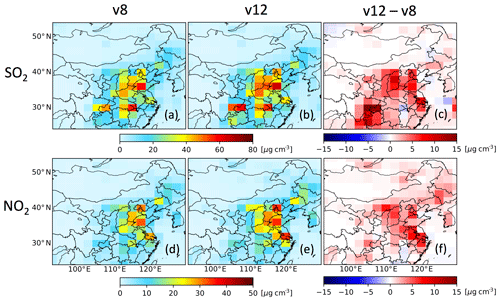Figure 1Simulations of surface SO2 and NO2 concentrations for October 2013. Panels (a) and (b) show surface SO2 simulated by GC adjoint v35m (developed based on GEOS-Chem version 8.2.1, updated through version 9, and we name it v8 for short) and GCv12.0.0 (v12 for short), respectively, and (c) shows the difference between v12 and v8. Panels (d)(f) are similar to (a)(c) but for NO2.

Download

## 3.2 Downscaling GEOS-Chem surface concentrations

GEOS-Chem surface SO2 and NO2 concentrations at a resolution of $\mathrm{2}{}^{\circ }×\mathrm{2.5}{}^{\circ }$ are not expected to be able to capture hot spots due to the CGS effect, and thus we aim to downscale them to finer resolutions. The prior emissions are MIX for October and November 2010. The posterior emissions are from separate inverse emission estimates in Part 1 (e.g., E−SO2 and E−NO2 experiments as described in Wang et al., 2020), unless it is specifically stated. The downscaling methods here should be distinguished from interpolation approaches to simply increasing spatial resolutions.

### 3.2.1 Downscaling concentrations with MIX simulations

With the assumption that surface concentrations of GEOS-Chem simulations using outdated emissions have correct spatial distributions at fine scales but systemic bias at coarse scales, we use $\mathrm{0.25}{}^{\circ }×\mathrm{0.3125}{}^{\circ }$ prior surface concentration patterns to downscale both prior and posterior $\mathrm{2}{}^{\circ }×\mathrm{2.5}{}^{\circ }$ simulations of surface species concentrations as shown in Eq. (1).

$\begin{array}{}\text{(1)}& {C}_{f,i}^{\mathrm{MIX}-\mathrm{DDC}}={C}_{\mathrm{c}}×\frac{{C}_{f,i}^{\mathrm{pri}}}{\frac{\mathrm{1}}{n}×{\sum }_{i=\mathrm{1}}^{n}{C}_{f,i}^{\mathrm{pri}}}\end{array}$

A coarse $\mathrm{2}{}^{\circ }×\mathrm{2.5}{}^{\circ }$ grid cell consists of n (64) fine $\mathrm{0.25}{}^{\circ }×\mathrm{0.3125}{}^{\circ }$ grid cells, and ${C}_{f,i}^{\mathrm{pri}}$ represents the MIX prior simulation of surface concentrations from the ith $\mathrm{0.25}{}^{\circ }×\mathrm{0.3125}{}^{\circ }$ grid cell within a $\mathrm{2}{}^{\circ }×\mathrm{2.5}{}^{\circ }$ grid cell. Thus, $\frac{\mathrm{1}}{n}×{\sum }_{i=\mathrm{1}}^{n}{C}_{f,i}^{\mathrm{pri}}$ is the mean $\mathrm{0.25}{}^{\circ }×\mathrm{0.3125}{}^{\circ }$ simulation in a $\mathrm{2}{}^{\circ }×\mathrm{2.5}{}^{\circ }$ grid cell, and $\frac{{C}_{f,i}^{\mathrm{pri}}}{\frac{\mathrm{1}}{n}×{\sum }_{i=\mathrm{1}}^{n}{C}_{f,i}^{\mathrm{pri}}}$ is the mass ratio between fine and coarse grids, which multiples the $\mathrm{2}{}^{\circ }×\mathrm{2.5}{}^{\circ }$ surface concentration, Cc, to obtain the downscaled result ${C}_{f,i}^{\mathrm{MIX}-\mathrm{DDC}}$. This approach is referred to as dynamic downscaling concentration with MIX simulation (MIX-DDC). Here, dynamic downscaling means the application of fine-scale model concentrations to downscale coarse-resolution concentrations.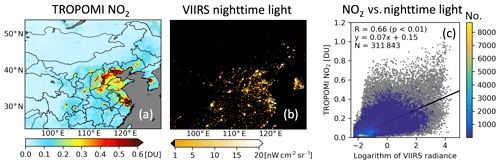Figure 2Panels (a) and (b) show TROPOMI NO2 VCD and VIIRS nighttime light radiance at the $\mathrm{0.05}{}^{\circ }×\mathrm{0.05}{}^{\circ }$ resolution in April 2018. Panel (c) shows a scatter plot of TROPOMI NO2 versus the logarithm of VIIRS nighttime light radiance (grid cells with VIIRS nighttime light radiance less than 0.1 nW cm−2 sr−1 are removed).

Download

### 3.2.2 Downscaling concentrations with nighttime lights

The VIIRS nighttime lights product at a resolution of $\mathrm{0.05}{}^{\circ }×\mathrm{0.05}{}^{\circ }$ is used to downscale GEOS-Chem simulations of surface NO2 due to its high spatial resolution and strong correlation with population distribution (Bennett and Smith, 2017) as well as NO2 VCDs. Figure 2a–b show the spatial distributions of VIIRS nighttime lights and TROPOMI NO2 tropospheric VCDs over China, and it is not surprising that both nighttime lights and NO2 hot spots are mainly over metropolises. Figure 2c shows a strong linear correlation between the logarithm of VIIRS nighttime lights and TROPOMI NO2 tropospheric VCDs at a resolution of $\mathrm{0.05}{}^{\circ }×\mathrm{0.05}{}^{\circ }$. This strong correlation is because (1) nighttime lights are a good spatial proxy for allocating NOx emissions (Geng et al., 2017); and (2) NO2 lifetime is short (several hours), which means the distribution of NO2 concentration hot spots is highly affected by source locations. This relationship is used to downscale as shown in Eq. (2) and (3).

$\begin{array}{}\text{(2)}& & {W}_{i}=\mathrm{ln}\left({V}_{i}\right)-\mathrm{ln}\left(\mathrm{0.1}\right)\text{(3)}& & {C}_{f,i}^{\mathrm{NL}-\mathrm{DC}}={C}_{\mathrm{c}}×\frac{{W}_{i}}{\stackrel{\mathrm{‾}}{W}}\end{array}$

Vi represents the i VIIRS $\mathrm{0.05}{}^{\circ }×\mathrm{0.05}{}^{\circ }$ nighttime light in a $\mathrm{2}{}^{\circ }×\mathrm{2.5}{}^{\circ }$ grid cell, and all nighttime lights less than 0.1 nW cm−2 sr−1 are set to be 0.1 nW cm−2 sr−1; thus, the minimum of Wi is naught. $\stackrel{\mathrm{‾}}{W}$ is the average of Wi in a $\mathrm{2}{}^{\circ }×\mathrm{2.5}{}^{\circ }$ grid cell, and we assume ${W}_{i}/\stackrel{\mathrm{‾}}{W}$ represents the ratio of the surface NO2 concentration at $\mathrm{0.05}{}^{\circ }×\mathrm{0.05}{}^{\circ }$ to that at $\mathrm{2}{}^{\circ }×\mathrm{2.5}{}^{\circ }$, due to the relationship between VIIRS nighttime lights and TROPOMI NO2 tropospheric VCDs. The ratio multiplies the surface NO2 concentration at $\mathrm{2}{}^{\circ }×\mathrm{2.5}{}^{\circ }$ Cc to obtain the downscaled result ${C}_{f,i}^{\mathrm{NL}-\mathrm{DC}}$. This approach is referred to as nighttime light downscaling concentration (NL-DC).

We do not expect that this approach can be used to downscale SO2 concentrations because (1) nighttime lights are not a very good spatial proxy for allocating SO2 emissions as SO2 emissions from traffic sector are very small, while nighttime lights are strong over rush traffic road; and (2) SO2 lifetime is 1–2 d, which is much longer than NO2 lifetime.

## 3.3 Downscaling emissions

To simulate or forecast surface SO2 and NO2 concentrations at a resolution of $\mathrm{0.25}{}^{\circ }×\mathrm{0.3125}{}^{\circ }$ through the GEOS-Chem model, the posterior emissions at a resolution of $\mathrm{2}{}^{\circ }×\mathrm{2.5}{}^{\circ }$ should be downscaled to fit the model resolution. The prior MIX 2010 emission inventory has a spatial resolution of $\mathrm{0.25}{}^{\circ }×\mathrm{0.25}{}^{\circ }$, which is slightly finer than $\mathrm{0.25}{}^{\circ }×\mathrm{0.3125}{}^{\circ }$, and it can be easily processed to fit $\mathrm{0.25}{}^{\circ }×\mathrm{0.3125}{}^{\circ }$ simulations through HEMCO – the GEOS-Chem emission processing package (Keller et al., 2014). Thus, all the posterior emissions at a resolution of $\mathrm{2}{}^{\circ }×\mathrm{2.5}{}^{\circ }$ are downscaled to $\mathrm{0.25}{}^{\circ }×\mathrm{0.25}{}^{\circ }$, which are further regridded to $\mathrm{0.25}{}^{\circ }×\mathrm{0.3125}{}^{\circ }$ with HEMCO. We introduce two emission downscaling approaches with prior MIX $\mathrm{0.25}{}^{\circ }×\mathrm{0.25}{}^{\circ }$ emissions and $\mathrm{0.05}{}^{\circ }×\mathrm{0.05}{}^{\circ }$ VIIRS nighttime lights used as spatial proxies. The two methods are referred to as downscaling emissions with MIX (MIX-DE) and downscaling emissions with nighttime light (NL-DE).

### 3.3.1 MIX-DE

We assume fine-resolution prior emission inventories have correct relative spatial distributions at fine scales, but a systemic bias exists at coarse scales. The emission downscaling approach is shown in Eq. (4), where ${E}_{f,i}^{\mathrm{pri}}$ is the ith MIX emission estimate at $\mathrm{0.25}{}^{\circ }×\mathrm{0.25}{}^{\circ }$ resolution in a $\mathrm{2}{}^{\circ }×\mathrm{2.5}{}^{\circ }$ grid cell for year 2010, n(=80) is the number of $\mathrm{0.25}{}^{\circ }×\mathrm{0.25}{}^{\circ }$ grids in a $\mathrm{2}{}^{\circ }×\mathrm{2.5}{}^{\circ }$ grid cell, ${E}_{\mathrm{c}}^{\mathrm{post}}$ is posterior emissions at $\mathrm{2}{}^{\circ }×\mathrm{2.5}{}^{\circ }$ from Wang et al. (2020), and ${E}_{f,i}^{\mathrm{MIX}-\mathrm{DE}}$ is the downscaled posterior emissions at $\mathrm{0.25}{}^{\circ }×\mathrm{0.25}{}^{\circ }$ resolution.

$\begin{array}{}\text{(4)}& {E}_{f,i}^{\mathrm{MIX}-\mathrm{DE}}={E}_{\mathrm{c}}^{\mathrm{post}}×\frac{{E}_{f,i}^{\mathrm{pri}}}{{\sum }_{i=\mathrm{1}}^{n}{E}_{f,i}^{\mathrm{pri}}}\end{array}$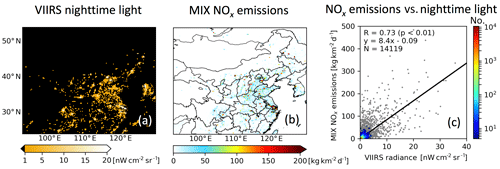Figure 3Panels (a) and (b) show VIIRS nighttime light radiance and MIX NOx emissions at $\mathrm{0.25}{}^{\circ }×\mathrm{0.25}{}^{\circ }$ resolution in April 2018 and April 2010, respectively. Panel (c) shows a scatter plot of MIX NOx emissions versus VIIRS nighttime light radiance (grid cells with VIIRS nighttime light radiance less than 0.1 nW cm−2 sr−1 are removed).

Download

### 3.3.2 NL-DE

VIIRS nighttime lights are good proxies for allocating CO2 emissions (Ou et al., 2015), and they are also expected to be useful for downscaling NOx emissions. Figure 3 shows that VIIRS nighttime lights and MIX NOx emissions have similar spatial patterns, and the linear correlation coefficient between them is as high as 0.73. Thus, VIIRS nighttime lights at a resolution of $\mathrm{0.05}{}^{\circ }×\mathrm{0.05}{}^{\circ }$ are used to downscale NOx emissions as shown in Eq. (5). ${E}_{\mathrm{c}}^{\mathrm{post}}$ represents posterior emissions at $\mathrm{2}{}^{\circ }×\mathrm{2.5}{}^{\circ }$ from Part 1 (Wang et al., 2020), Ai and Vi are the ith area and VIIRS nighttime lights at $\mathrm{0.05}{}^{\circ }×\mathrm{0.05}{}^{\circ }$, respectively, and n(=2000) is the number of $\mathrm{0.05}{}^{\circ }×\mathrm{0.05}{}^{\circ }$ grids in a $\mathrm{2}{}^{\circ }×\mathrm{2.5}{}^{\circ }$ grid cell. ${E}_{f,i}^{\mathrm{NL}-\mathrm{DE}}$ is the downscaled posterior NOx emissions at $\mathrm{0.05}{}^{\circ }×\mathrm{0.05}{}^{\circ }$, which is further aggregated to $\mathrm{0.25}{}^{\circ }×\mathrm{0.25}{}^{\circ }$.

$\begin{array}{}\text{(5)}& {E}_{f,i}^{\mathrm{NL}-\mathrm{DE}}={E}_{\mathrm{c}}^{\mathrm{post}}×\frac{{A}_{i}{V}_{i}}{{\sum }_{i=\mathrm{1}}^{n}{A}_{i}{V}_{i}}\end{array}$

Table 1Design of experiments for simulating surface SO2 and NO2 concentrations over China in October 2013.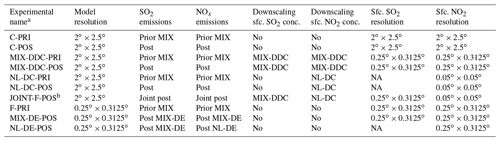a The nomenclature of the experimental name is as follows. C: coarse resolution, F: fine resolution, PRI: prior, POS: posterior, DDC: dynamic downscaling concentration, DC: downscaling concentration, NL: nighttime light, MIX: prior MIX emission inventory, DE: downscaling emissions, JOINT: emission inventory from joint inverse modeling. b JOINT-F-POS is a set of experiments using posterior emission inventories from joint inversion modeling using a different observation balance parameter γ.

## 3.4 Design of experiments

### 3.4.1 Simulations for October 2013

A set of GEOS-Chem simulation experiments are designed to illustrate the impacts of model resolutions and emission inventories on simulating surface SO2 and NO2 concentrations over China for October 2013, as summarized in Table 1. Simulations of surface SO2 and NO2 concentrations are evaluated with in situ observations. C-PRI and C-POS are designed to show the CGS effect of surface SO2 and NO2 concentrations in coarse (C-) resolution simulations with prior (PRI) and posterior (POS) emissions, respectively. Both C-PRI and C-POS have a simulation resolution of $\mathrm{2}{}^{\circ }×\mathrm{2.5}{}^{\circ }$ and use the prior and posterior emissions, respectively. MIX-DDC-PRI, MIX-DDC-POS, NL-DC-PRI, and NL-DC-POS illustrate alleviation of the CGS effect through downscaling of surface concentrations. In MIX-DDC-PRI and MIX-DDC-POS, surface SO2 and NO2 concentrations at $\mathrm{2}{}^{\circ }×\mathrm{2.5}{}^{\circ }$ from C-PRI and C-POS are downscaled to the resolution of $\mathrm{0.25}{}^{\circ }×\mathrm{0.3125}{}^{\circ }$ through the MIX-DDC approach. NL-DC-PRI and NL-DC-POS downscale NO2 concentrations at $\mathrm{2}{}^{\circ }×\mathrm{2.5}{}^{\circ }$ from C-PRI and C-POS to the resolution of $\mathrm{0.05}{}^{\circ }×\mathrm{0.05}{}^{\circ }$ through the NL-DC approach. JOINT-F-POS is designed to show the impacts of using posterior emissions from joint (JOINT) assimilations on surface SO2 and NO2 forecast at fine (F) spatial scale. In JOINT-F-POS, posterior SO2 and NOx emissions from joint assimilations with various observation balance parameter (γ) values from Part 1, i.e., Wang et al. (2020), are used to simulate surface SO2 and NO2 at $\mathrm{2}{}^{\circ }×\mathrm{2.5}{}^{\circ }$; this parameter is used to balance the importance of the SO2 and NO2 observational terms in the cost function. The simulated surface SO2 and NO2 concentrations are further downscaled to $\mathrm{0.25}{}^{\circ }×\mathrm{0.3125}{}^{\circ }$ through the MIX-DDC approach and $\mathrm{0.05}{}^{\circ }×\mathrm{0.05}{}^{\circ }$ through the NL-DC approach, respectively. F-PRI, MIX-DE-POS, and NL-DE-POS illustrate the improvements when using downscaled posterior emissions to simulate surface SO2 and NO2 concentrations. All three simulations have a resolution of $\mathrm{0.25}{}^{\circ }×\mathrm{0.3125}{}^{\circ }$ but use different emission inventories. F-PRI uses the prior MIX emissions, but MIX-DE-POS and NL-DE-POS use the downscaled posterior emissions. Posterior SO2 emissions downscaled through the MIX-DE approach are used in the two simulations, but posterior NOx emissions used in MIX-DE-POS and NL-DE-POS are downscaled through the MIX-DE and NL-DE approaches, respectively.

Table 2Design of experiments for forecasting surface SO2, NO2, and O3 concentrations over China in November 2013.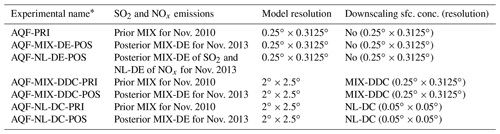* The nomenclature of the experimental name is as follows. AQF: air quality forecasts, PRI: prior, POS: posterior, MIX: prior MIX emission inventory, NL: nighttime light, DE: downscaling emissions, DDC: dynamic downscaling concentration, DC: downscaling concentration.

### 3.4.2 Forecasts for November 2013

Y. Wang et al. (2016) used posterior emissions of the current month to improve air quality forecasts of the next month. We implement a similar approach in this study, but emission variations among different months are also considered. With the assumption that the prior MIX emission inventory has proper temporal variations among different months, posterior MIX-DE and NL-DE emission inventories for November 2013 are obtained by multiplying posterior MIX-DE or NL-DE emission inventories for October 2013, respectively, by the ratios of prior MIX emissions between November and October 2010. As summarized in Table 2, we design a set of experiments for air quality forecasts (AQFs) of surface SO2, NO2, and O3 concentrations at fine resolution over China in November 2013. AQF-PRI uses the prior MIX inventory for November 2010 to forecast surface SO2, NO2, and O3 concentrations of November 2013 at $\mathrm{0.25}{}^{\circ }×\mathrm{0.3125}{}^{\circ }$, while AQF-MIX-DE-POS uses the posterior MIX-DE inventory for November 2013. AQF-NL-DE-POS is similar to AQF-MIX-DE-POS, but the posterior NL-DE inventory for NOx is used. AQF-MIX-DDC-PRI and AQF-MIX-DDC-POS use the prior MIX for November 2010 and posterior MIX-DE for November 2013 inventories to forecast surface SO2 and NO2 concentrations at $\mathrm{2}{}^{\circ }×\mathrm{2.5}{}^{\circ }$, which are further downscaled to $\mathrm{0.25}{}^{\circ }×\mathrm{0.3125}{}^{\circ }$ through the MIX-DDC approach. Since NO2 hot spots cannot be captured at $\mathrm{0.25}{}^{\circ }×\mathrm{0.3125}{}^{\circ }$ resolution, the NL-DC approach is also applied to the NO2 forecasts. Thus, AQF-NL-DC-PRI and AQF-NL-DC-POS use the prior MIX inventory for November 2010 and the posterior MIX-DE inventory for November 2013 to forecast surface SO2 and NO2 concentrations of November 2013 at $\mathrm{2}{}^{\circ }×\mathrm{2.5}{}^{\circ }$, which are further downscaled to $\mathrm{0.05}{}^{\circ }×\mathrm{0.05}{}^{\circ }$ according to VIIRS nighttime light of October 2013 through the NL-DC approach.

## 3.5 Evaluation statistics

We use the linear correlation coefficient (R), mean bias (MB), normalized mean bias (NMB), normalized centered root mean square error (NCRMSE) (Wang et al., 2020), and normalized mean squared error (NMSE) as measures to evaluate GEOS-Chem SO2 and NO2 surface concentrations with in situ observations. NCRMSE measures the spatial distribution difference between forecasts, and in situ observations are similar to the root mean squared error, but the impact of bias is removed. NMSE is defined as Eq. (6), where Mi and Oi are the ith GEOS-Chem simulation and in situ observation, respectively, $\stackrel{\mathrm{‾}}{O}$ is mean of the observations, and N is number of the observations.

$\begin{array}{}\text{(6)}& \mathrm{NMSE}=\frac{\frac{\mathrm{1}}{N}{\sum }_{i=\mathrm{1}}^{N}\left({M}_{i}-{O}_{i}{\right)}^{\mathrm{2}}}{\frac{\mathrm{1}}{N}{\sum }_{i=\mathrm{1}}^{N}\left({O}_{i}-\stackrel{\mathrm{‾}}{O}{\right)}^{\mathrm{2}}}\end{array}$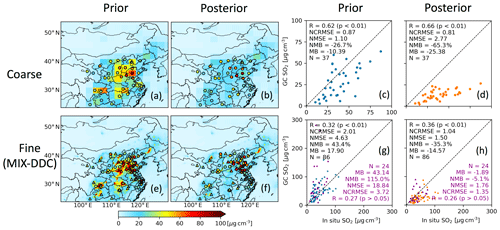Figure 4Evaluations of coarse-resolution, MIX-DDC GEOS-Chem surface SO2 simulations with in situ observations for October 2013. Panels (a) and (b) show C-PRI and C-POS simulations, respectively, with in situ observations overlapped. If there is more than one observation in a $\mathrm{2}{}^{\circ }×\mathrm{2.5}{}^{\circ }$ grid cell, they are averaged. Panels (c) and (d) show scatter plots of C-PRI and C-POS simulations versus in situ observations, respectively. Panels (e) and (f) show surface SO2 concentrations of MIX-DDC-PRI and MIX-DDC-POS, respectively, with in situ province-capital-city (triangle) and non-province-capital-city (circle) observations overlapped. Panels (g) and (h) show scatter plots of MIX-DDC-PRI and MIX-DDC-POS simulations versus in situ province-capital-city (triangle) and non-province-capital-city (circle) observations, respectively. The linear correlation coefficient (R), normalized centered root mean squared error (NCRMSE), normalized mean squared error (NMSE), normalized mean bias (NMB), mean bias (MB), and number of observations (N) are shown over scatter plots, with black color text for all observations and purple color text for province-capital-city observations.

Download

4 Results

## 4.1 CGS and MIX-DDC for SO2

The CGS effect of surface SO2 concentrations in the coarse-resolution ($\mathrm{2}{}^{\circ }×\mathrm{2.5}{}^{\circ }$) simulations (C-PRI and C-POS experiments) is shown in Fig. 4a–d. The GEOS-Chem $\mathrm{2}{}^{\circ }×\mathrm{2.5}{}^{\circ }$ simulation of every grid cell is the average of surface SO2 at $\sim \mathrm{5}×{\mathrm{10}}^{\mathrm{4}}$ km2 area, while in situ SO2 observations can only represent average concentrations of a much smaller area. Considering that all sites are in cities, where emission sources are located, GEOS-Chem $\mathrm{2}{}^{\circ }×\mathrm{2.5}{}^{\circ }$ simulations are expected to be smaller than in situ observations. In this study, the NMB is −26.7 % (Fig. 4c) in the C-PRI simulation, while the C-POS simulation shows an even stronger negative NMB of bias of −65.3 % (Fig. 4d), as the posterior SO2 emission is 35.8 % smaller than prior MIX 2010.

To decrease the impact of CGS on surface SO2 simulations, both the prior and posterior GEOS-Chem surface SO2 simulations at $\mathrm{2}{}^{\circ }×\mathrm{2.5}{}^{\circ }$ resolution are downscaled to $\mathrm{0.25}{}^{\circ }×\mathrm{0.3125}{}^{\circ }$ through the MIX-DDC approach (MIX-DDC-PRI and MIX-DDC-POS experiments). Zheng et al. (2017) showed that surface SO2 concentration simulations from WRF-CMAQ, when evaluating with in situ observations, have a NMB of −23 %, 7 %, and 41 % at the resolutions of 36 km ( 0.36), 12 km ( 0.12), and 4 km ( 0.04), respectively, which suggests that (1) the CGS effect and other nonlinear resolution-dependent processes can affect SO2 simulation results and (2) these problems are alleviated at the resolution of $\mathrm{0.25}{}^{\circ }×\mathrm{0.3125}{}^{\circ }$ but are not completely avoided. The downscaled prior (MIX-DDC-PRI) and posterior (MIX-DDC-POS) GEOS-Chem surface SO2 concentrations at $\mathrm{0.25}{}^{\circ }×\mathrm{0.3125}{}^{\circ }$ are shown in Fig. 4e–h. MIX-DDC-PRI and MIX-DDC-POS SO2 simulations show hot spots of up to 270 µg m−3 (Fig. 4e) and 80 µg m−3 (Fig. 4f), respectively, compared with the largest value of less than 60 µg m−3 (Fig. 4a) and 35 µg m−3 (Fig. 4b) in the C-PRI and C-POS simulations, respectively.

MIX-DDC-POS SO2 simulations are in better agreement with in situ observations than MIX-DDC-PRI. The NMSE decreases from 4.63 in MIX-DDC-PRI to 1.50 in MIX-DDC-POS, and the linear correlation coefficient (R) increases from 0.32 to 0.36 (Fig. 4g–h). The NMB changes, however, from 43.4 % to −35.3 % (Fig. 3g–h), which implies the CGS effect may not be completely avoided at a resolution of $\mathrm{0.25}{}^{\circ }×\mathrm{0.3125}{}^{\circ }$, and other factors that may affect the result should be investigated in the future. We also separately compare MIX-DDC-PRI and MIX-DDC-POS simulations with in situ observations over provincial capital cities, as SO2 hot spots in smaller cities may still be difficult to capture using the $\mathrm{0.25}{}^{\circ }×\mathrm{0.3125}{}^{\circ }$ MIX-DDC-PRI and MIX-DDC-POS simulations. The NMB is 115.0 % in the MIX-DDC-PRI simulation and reduces to −5 % in the MIX-DDC-POS simulation. Additionally, the MIX-DDC-POS simulation shows better spatial pattern than the MIX-DDC-PRI simulation in terms of NCRMSE, although linear correlation decreases slightly. In spite of the improvement of capturing hot spots in term of NMB using the MIX-DDC approach, we should also note that the coarse-resolution simulations (Fig. 4c, d) have larger linear correlation coefficients and smaller NCRMSEs than the MIX-DDC simulations. Thus, for SO2 simulations, MIX-DDC helps to capture hot spots but can make the spatial distribution worse than the original coarse-resolution simulations in terms of NCRMSE. The spatial pattern degradation implies that current chemistry transport simulations of surface SO2 concentrations can capture the regional spatial pattern (coarse resolution) well, but it is difficult to simulate the local spatial pattern (fine resolution); the weakness for describing the local spatial pattern simulation suggests the uncertainties of either bottom-up SO2 emission estimates at fine resolution or locally resolved meteorological fields (Ge et al., 2017), or both. This uncertainty in bottom-up emission inventories can further stem from distributing SO2 emissions at the provincial level to a fine-resolution grid. MIX-DDC also performs well when GC adjoint v35m is used for coarse resolution, and results show better spatial pattern (smaller NCRMSE) than using GC v12.0.0 (Fig. S2).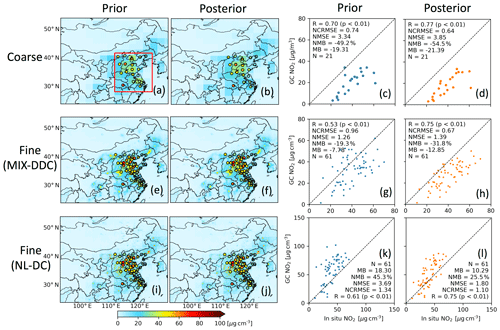Figure 5Evaluation of coarse-resolution, MIX-DDC, and NL-DC GEOS-Chem surface NO2 simulations with in situ observations for October 2013. Panels (a) and (b) show C-PRI and C-POS simulations, respectively, with in situ observations overlapped. If there is more than one observation in a $\mathrm{2}{}^{\circ }×\mathrm{2.5}{}^{\circ }$ grid cell, they are averaged. Panels (c) and (d) show scatter plots of C-PRI and C-POS simulations versus in situ observations, respectively. Panels (e) and (f) show GEOS-Chem surface NO2 of MIX-DDC-PRI and MIX-DDC-POS, respectively, with in situ observations overlapped. Panels (g) and (h) show scatter plots of MIX-DDC-PRI and MIX-DDC-POS simulations versus in situ observations, respectively. Panels (i)(l) are similar to (e)(h), respectively, but results are downscaled through the NL-DC approach (NL-DC-PRI and NL-DC-POS). The linear correlation coefficient (R), normalized centered root mean squared error (NCRMSE), normalized mean squared error (NMSE), normalized mean bias (NMB), mean bias (MB), and number of observations (N) are shown over scatter plots. As NOx emission is mainly over the North China Plain and eastern China, validation with in situ surface NO2 is conducted at these areas (red box in a).

Download

## 4.2 CGS, MIX-DDC, and NL-DC for NO2

NO2 has an even shorter lifetime than SO2; thus the CGS effect also exists in the C-PRI and C-POS simulations. Figure 5a–d show that almost all in situ NO2 observations are larger than the GEOS-Chem simulations, regardless of whether the prior MIX 2010 (C-PRI) or the posterior (C-POS) NOx emissions are used. GEOS-Chem surface NO2 averages from the C-PRI and C-POS simulations, sampled according to in situ observational sites, are 49.2 % and 54.5 % smaller than the average of in situ observations, respectively.

The MIX-DDC approach is also applied to downscale NO2 surface simulations (MIX-DDC-PRI and MIX-DDC-POS experiments), and the results are evaluated with in situ observations. As discussed, according to Zheng et al. (2017), the CGS effect and other nonlinear resolution-dependent problems can be alleviated at the resolution of $\mathrm{0.25}{}^{\circ }×\mathrm{0.3125}{}^{\circ }$ (such as in MIX-DDC-PRI and MIX-DDC-POS experiments) but cannot be completely avoided. The MIX-DDC-POS simulation is better than the MIX-DDC-PRI simulation in terms of spatial pattern (NCRMSE), although the CGS effect may still exists and other nonlinear resolution-dependent problem should be investigated in the future. The NMB is −19.3 % and −31.8 % for the MIX-DDC-PRI and MIX-DDC-POS simulations, respectively (Fig. 5e–h), which implies that $\mathrm{0.25}{}^{\circ }×\mathrm{0.3125}{}^{\circ }$ may be still too coarse to capture hot spots due to the short lifetime of NO2. The larger negative bias in the MIX-DDC-POS simulation than in the MIX-DDC-PRI also leads to the former showing large NMSE. Despite the negative bias, R between observations and the MIX-DDC simulations increases from 0.53 in MIX-DDC-PRI to 0.75 in MIX-DDC-POS, and the NCRMSE reduces from 0.96 to 0.67, which is only slightly larger than 0.64 in the C-POS simulation. Thus MIX-DDC-POS can better capture NO2 hot spots and shows spatial pattern as good as C-POS. MIX-DDC also performs well when GC adjoint v35m is used for coarse-resolution simulations, and results show better spatial pattern (smaller NCRMSE) than using GC v12.0.0 (Fig. S3).

To further alleviate the CGS effect, we downscale GEOS-Chem surface NO2 simulations at $\mathrm{2}{}^{\circ }×\mathrm{2.5}{}^{\circ }$ to $\mathrm{0.05}{}^{\circ }×\mathrm{0.05}{}^{\circ }$ according to VIIRS nighttime light distributions through the NL-DC approach (NL-DC-PRI and NL-DC-POS experiments), and the results are evaluated with in situ surface NO2 observations (Fig. 5i–l). The largest GEOS-Chem surface NO2 values are less than 35 µg m−3 in both the coarse C-PRI and C-POS simulations (Fig. 5a, b), while they are larger than 100 µg m−3 at $\mathrm{0.05}{}^{\circ }×\mathrm{0.05}{}^{\circ }$ in the NL-DC-PRI and NL-DC-POS simulations (Fig. 5i, j). The scatter plots of the NL-DC-PRI (Fig. 5k) and NL-DC-POS (Fig. 5l) simulations versus in situ surface NO2 observations show that R increases from 0.61 in the NL-DC-PRI simulation to 0.75 in the NL-DC-POS simulation, and NMSE decreases from 3.69 to 1.80, which is smaller than that in the coarse-resolution simulations and the MIX-DDC downscaled simulation. It suggests that NL-DC has the advantage of downscaling surface concentrations (without evoking any CTM simulation and its associated needs of computational resources). The biases of surface NO2 concentrations are 45.3 % and 25.5 % for NL-DC-PRI and NL-DC-POS, respectively, which could come from total emission bias as well as the downscaling process through the NL-DC approach. The sites used for validation are mainly over urban regions, and we lack sites that are located over rural regions to evaluate if positive or negative bias persists over rural regions. Thus, we are not able to determine how much of the positive bias in NL-DC-PRI and NL-DC-POS is caused by the NL-DC approach. When NL-DC is applied to downscale GC adjoint v35m coarse-resolution simulations, results show better spatial pattern (smaller NCRMSE) than that of GC v12.0.0 (Fig. S3).

The surface NO2 concentrations used for evaluation are derived from measurements of (NO2)m assuming r${}_{{\mathrm{NO}}_{\mathrm{2}}}$ of 0.83 as stated in Sect. 2.1. Due to the lack of information on r${}_{{\mathrm{NO}}_{\mathrm{2}}}$, we also test the values in the range of 0.7 to 1.0, and the derived NO2 concentrations are used to validate the NL-DC-PRI and NL-DC-POS simulations at the $\mathrm{0.05}{}^{\circ }×\mathrm{0.05}{}^{\circ }$ resolution. Figure 6 shows that the NL-DC-POS simulation has NMSE in the range of 1 to 4, which is always better than the NL-DC-PRI simulation with NMSE in the range of 2 to 8.Figure 6Normalized mean squared error (NMSE) for NL-DC-PRI (blue line) and NL-DC-POS (orange line) when evaluating with in situ surface NO2 derived from various NO2∕(NO2)m ratios (increasing from 0.7 to 1.0 with a step of 0.01), where (NO2)m is measured NOz concentration.

Download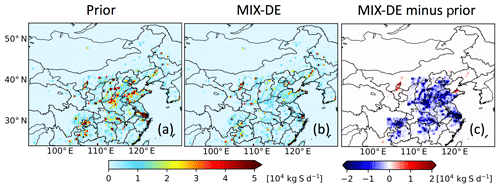Figure 7Panel (a) shows SO2 $\mathrm{0.25}{}^{\circ }×\mathrm{0.3125}{}^{\circ }$ emissions of prior MIX 2010, (b) shows posterior MIX-DE, and (c) shows the difference between posterior MIX-DE and prior MIX 2010.

Download

## 4.3 MIX-DE for SO2 simulations

Instead of downscaling simulation results as shown in Sect. 4.1, we directly simulate surface SO2 concentrations at $\mathrm{0.25}{}^{\circ }×\mathrm{0.3125}{}^{\circ }$ resolution through GEOS-Chem over China in October 2013 using the prior MIX 2010 emissions and the posterior emissions. The posterior SO2 emissions at $\mathrm{2}{}^{\circ }×\mathrm{2.5}{}^{\circ }$ resolution are downscaled to $\mathrm{0.25}{}^{\circ }×\mathrm{0.3125}{}^{\circ }$ through the MIX-DE approach. The posterior MIX-DE SO2 emissions are smaller than the prior MIX 2010 SO2 emissions over northern China and southwestern China (Fig. 7).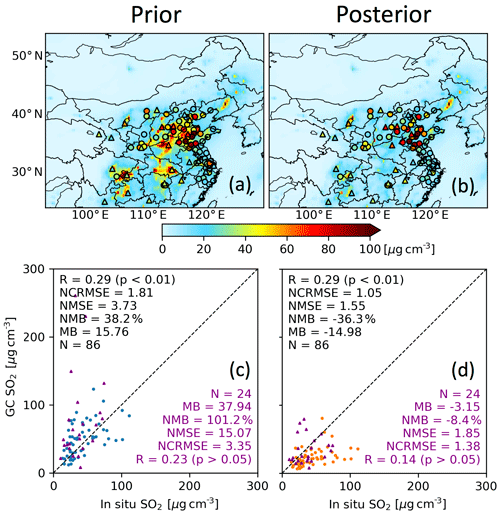Figure 8Evaluations of fine-resolution GEOS-Chem surface SO2 simulations with in situ observations for October 2013. Panels (a) and (b) show surface SO2 concentrations of F-PRI and MIX-DE-POS, respectively, with in situ province-capital-city (triangle) and non-province-capital-city (circle) observations overlapped. Panels (c) and (d) show scatter plots of F-PRI and MIX-DE-POS simulations versus in situ province-capital-city (triangle) and non-province-capital-city (circle) observations, respectively. The linear correlation coefficient (R), normalized centered root mean squared error (NCRMSE), normalized mean squared error (NMSE), normalized mean bias (NMB), mean bias (MB), and number of observations (N) are shown over scatter plots, with black color text for all observations and purple color text for province-capital-city observations.

Download

The $\mathrm{0.25}{}^{\circ }×\mathrm{0.3125}{}^{\circ }$ GEOS-Chem simulations of surface SO2 for October 2013 when using the prior MIX (F-PRI experiment) and the posterior MIX-DE (MIX-DE-POS experiment) emission inventories are shown in Fig. 8. When evaluating with all in situ SO2 observations, NMSE decreases from 3.73 in F-PRI to 1.55 in MIX-DE-POS, but bias changes from 15.76 to −14.98µg m−3. For the same reason, in the MIX-DDC-PRI and MIX-DDC-POS assessment in Sect. 4.1, we also focus on provincial capital cities. In this scene, NMSE of the MIX-DE-POS simulation is 1.85 (Fig. 8d), which is much smaller than 15.07 in the F-PRI simulation (Fig. 8c), but it is slightly larger than 1.76 in the MIX-DDC-POS simulation (Fig. 4h). Moreover, NMB decreases from 101.2 % in the F-PRI simulation to −8.4 % in the MIX-DE-POS simulation (Fig. 8). R is 0.23 and 0.14 in F-PRI and MIX-DE-POS, respectively, neither of which is significant at the 95 % confidence level. In Sect. 4.2, we have shown that good spatial distribution is captured in coarse-resolution rather than fine-resolution simulations, which implies that large uncertainty of bottom-up SO2 emission estimates at fine resolution may be introduced when distributing SO2 emissions at the provincial level to a fine-resolution grid. It is not surprising that the correlation coefficients are small for F-PRI and MIX-DE-POS, as both are in fine resolution.

## 4.4 MIX-DE and NL-DE for NO2 simulations

Posterior NOx emissions at $\mathrm{2}{}^{\circ }×\mathrm{2.5}{}^{\circ }$ resolution are downscaled through MIX-DE and NL-DE approaches. Figure 9 shows the prior MIX, posterior MIX-DE, and posterior NL-DE NOx emissions at $\mathrm{0.25}{}^{\circ }×\mathrm{0.3125}{}^{\circ }$ resolutions. All three emission inventories show NOx emission hot spots over metropolises (Fig. 9a–c). Compared with prior MIX, posterior MIX-DE is larger over Hebei Province but smaller over most other areas of the North China Plain and eastern China (Fig. 9d). As the posterior NL-DE emission inventory is downscaled according to the VIIRS nighttime light distribution, the difference (Fig. 9e) between posterior NL-DE and prior MIX and the difference (Fig. 9f) between posterior NL-DE and posterior MIX-DE show scattered positive and negative values.

The three emission inventories are used to simulate surface NO2 concentrations at the $\mathrm{0.25}{}^{\circ }×\mathrm{0.3125}{}^{\circ }$ resolution over China in October 2013, that is F-PRI, MIX-DE-POS, and NL-DE-POS experiments in Table 1. All these simulations are evaluated with in situ NO2 observations (Fig. 10). R increases from 0.46 in F-PRI to 0.61 in MIX-DE-POS and 0.58 in NL-DE-POS, and NCRMSE decreases from 0.95 in F-PRI to 0.81 in MIX-DE-POS and 0.85 in NL-DE-POS (Fig. 10d–f). Both MIX-DE-POS and NL-DE-POS show stronger negative NMB and larger NMSE than F-PRI, which should be partly caused by the CGS effect, although emission bias and other nonlinear resolution-dependent processes could play a role.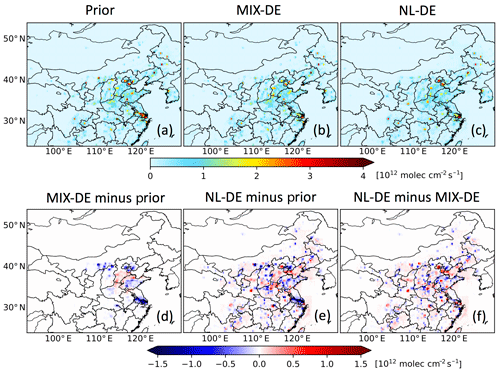Figure 9NOx $\mathrm{0.25}{}^{\circ }×\mathrm{0.3125}{}^{\circ }$ emissions of prior MIX 2010 (a), posterior MIX-DE (b), posterior NL-DE (c), the difference between posterior MIX-DE and prior MIX 2010 (d), the difference between posterior NL-DE and prior MIX 2010 (e), and the difference between posterior NL-DE and posterior MIX-DE (f).

Download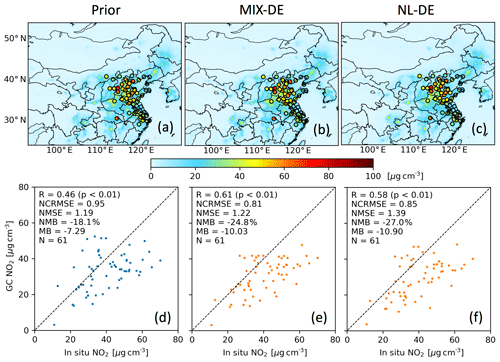Figure 10Evaluations of fine-resolution GEOS-Chem surface NO2 simulations with in situ observations for October 2013. Panels (a)(c) show surface NO2 concentrations of F-PRI, MIX-DE-POS, and NL-DE-POS, respectively, with in situ observations overlapped. Panels (d)(f) show scatter plots of F-PRI, MIX-DE-POS, and NL-DE-POS simulations versus in situ observations, respectively. The linear correlation coefficient (R), normalized centered root mean squared error (NCRMSE), normalized mean squared error (NMSE), normalized mean bias (NMB), mean bias (MB), and number of observations (N) are shown over scatter plots.

Download

MIX-DE-POS has improved values of R and NCRMSE compared to NL-DE-POS; here we discuss the possible reasons and propose future works to improve NL-DE. MIX is a mosaic bottom-up emission inventory, and it is actually the MEIC emission inventory for NOx emissions over China (Li et al., 2017a). The MIX (or MEIC) NOx emission inventory over China consists of emissions from four sectors including coal-fired power plant, industrial, transport, and residential sectors. Coal-fired power plant emissions in MEIC are derived through extensively using detailed information (including locations of individual units) of 7657 generation units in China (Liu et al., 2015); coal-fired power plant emissions can be accurately placed in grids according to source location information (Li et al., 2017a). Thus, if we can allocate posterior total anthropogenic NOx emissions into the four sectors, we expect that it is better to use the MIX coal-fired power plant NOx emission inventory rather than nighttime lights to downscale the posterior coal-fired power plant NOx emissions. For the other sectors in MIX (or MEIC) over China, population density is used to allocate industrial and residential emissions to grids (Li et al., 2017a), and transport emissions are distributed according to road networks (Li et al., 2017a). Using population density to downscale industrial and residential NOx could underestimate emissions over urban regions, compared with the approach using nighttime light which could better represent economic development levels (Geng et al., 2017). Whether it is better to use road networks or nighttime lights to downscale NOx emissions from the transport sector requires future investigations. In this study, the posterior NOx emission inventory to be downscaled is total anthropogenic NOx emissions, which is not allocated into different source sectors. Thus, if we assume that the ratios of every sectoral emissions to total anthropogenic emissions do not change between prior and posterior emission inventories, MIX-DE has an advantage for the coal-fired power sector, while NL-DE could benefit the downscaling for the industrial and residential sectors. In future work, we could optimize sectoral emissions rather than total anthropogenic emissions, subsequently downscale posterior coal-fired power emissions through prior MIX coal-fired power emissions, and ultimately use VIIRS nighttime light data to downscale posterior industrial and residential emissions.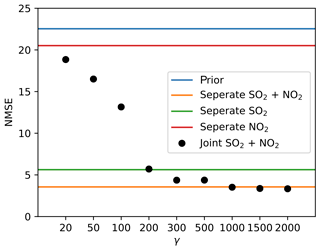Figure 11Sum of normalized mean squared error (NMSE) of surface SO2 and NO2. All surface SO2 and NO2 simulations come from MIX-DDC and NL-DC, respectively. Black dots are posterior simulations from Joint-F-POS. The blue line shows prior simulation results with SO2 NMSE from MIX-DDC-PRI and NO2 NMSE from NL-DC-PRI. The orange line shows simulation results with SO2 NMSE from MIX-DDC-POS and NO2 NMSE from NL-DC-POS. The green line is similar to the orange line, but the posterior SO2 emission from separate assimilation and prior NOx emission are used. The red line is similar to the orange line, but the posterior NOx emission from separate assimilation and prior SO2 emission are used.

Download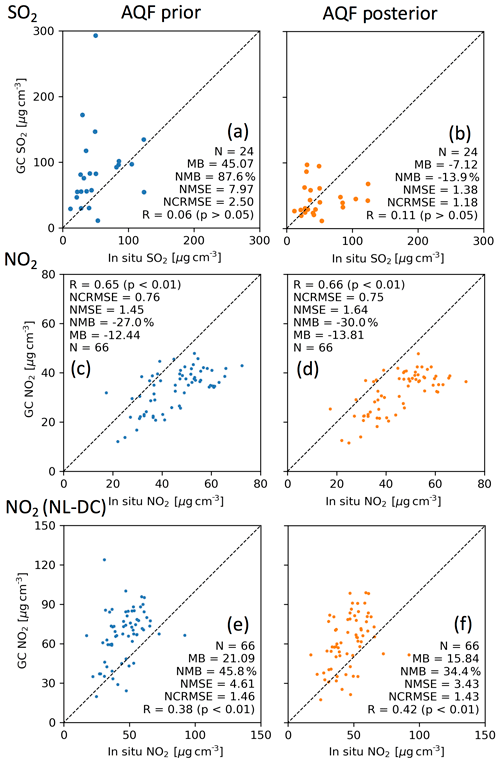Figure 12Evaluation of GEOS-Chem surface SO2 and NO2 forecasts with in situ observations for November 2013. Panels (a) and (b) show scatter plots of AQF-PRI and AQF-MIX-DE-POS SO2 at $\mathrm{0.25}{}^{\circ }×\mathrm{0.3125}{}^{\circ }$ versus in situ province-capital-city observations, respectively. Panels (c) and (d) show scatter plots of AQF-PRI and AQF-MIX-DE-POS NO2 at $\mathrm{0.25}{}^{\circ }×\mathrm{0.3125}{}^{\circ }$ versus in situ observations, respectively. Panels (e) and (f) show scatter plots of AQF-NL-DC-PRI and AQF-NL-DC-POS NO2 at $\mathrm{0.05}{}^{\circ }×\mathrm{0.05}{}^{\circ }$ versus in situ observations, respectively. The linear correlation coefficient (R), normalized centered root mean squared error (NCRMSE), normalized mean squared error (NMSE), normalized mean bias (NMB), mean bias (MB), and number of observations (N) are shown over scatter plots.

Download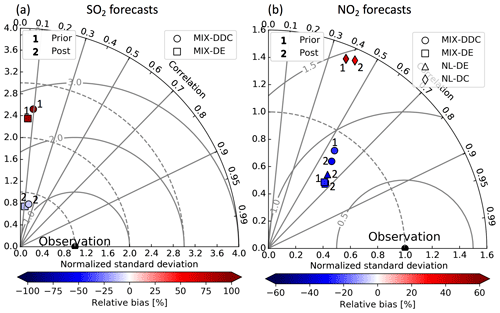Figure 13Taylor diagrams of evaluations of surface SO2 (a) and NO2 (b) forecasts with in situ observations. Circle 1 represents AQF-MIX-DDC-PRI, circle 2 AQF-MIX-DDC-POS, square 1 AQF-PRI, square 2 AQF-MIX-DE-POS, triangle 2 AQF-NL-DE-POS, diamond 1 AQF-NL-DC-PRI, and diamond 2 AQF-NL-DC-POS.

Download

## 4.5 Impacts of joint assimilations on surface SO2 and NO2 simulations

To evaluate the posterior SO2 and NOx emissions of joint assimilations with various observation balance parameter (γ) values from Part 1, i.e., Wang et al. (2020), we focus on the sum of NMSE of surface SO2 and NO2 as shown in Fig. 11. The experiment using the prior MIX SO2 and NOx emissions has the largest sum of NMSE, which is followed by the simulation using the prior MIX SO2 emissions and the posterior NOx emissions from separate assimilation. The sum of NMSE using the posterior SO2 and NOx emissions of joint assimilations (JOINT-F-POS) with various observation balance parameter (γ) values (as γ increases, the NO2 species is more emphasized in the cost function) is always smaller than that of the experiment using the prior MIX SO2 emissions and the posterior NOx emission from separate assimilation and decreases as γ increases. When γ is 1500 or 2000, the sum of NMSE using the posterior SO2 and NOx emissions of joint assimilations is smaller than that of the experiment using the prior MIX NOx emission and the posterior SO2 emission from separate assimilation, but it equals that of the experiment using the posterior SO2 and NOx emissions from separate assimilations. The value of γ mainly affects SO2 NMSE (Fig. S4a) rather than NO2 NMSE (Fig. S4b).

## 4.6 Application for forecasts

Figure 12 shows evaluations of surface SO2 and NO2 forecasts with in situ observations. AQF-PRI SO2 concentrations are generally larger than in situ observations with MB of 45.07 µg m−3 and NMSE of 7.97 (Fig. 12a). The MB and NMSE reduces to −7.12µg m−3 and 1.38 (Fig. 12b), respectively, in AQF-MIX-DE-POS. For surface NO2, NCRMSE and R are 0.76 and 0.65 (Fig. 12c), respectively, in AQF-PRI and change to 0.75 and 0.66 (Fig. 12d), respectively, in AQF-MIX-DE-POS. The stronger negative NMB and larger NMSE for NO2 in AQF-MIX-DE-POS than that in AQF-PRI are likely attributable to the CGS effect. The CGS effect is eliminated in both the AQF-NL-DC-PRI and AQF-NL-DC-POS, which show positive bias (Fig. 12e, f). In the $\mathrm{0.05}{}^{\circ }×\mathrm{0.05}{}^{\circ }$ forecasts, NMSE decreases from 4.61 in AQF-NL-DC-PRI to 3.43 in AQF-NL-DC-POS, and R increases from 0.38 to 0.42.

In this study, we show the improvements of GEOS-Chem simulations or forecasts of surface SO2 and NO2 concentrations through posterior emissions constrained by integration of GEOS-Chem adjoint and OMPS observations. All the improvements of SO2 and NO2 forecasts are summarized in the Taylor diagrams (Fig. 13), which includes R, normalized standard deviation (the ratio of forecast standard deviation to in situ observations), NMB, and normalized centered root mean square error (NCRMSE). NCRMSE is shown as the distance between the forecast point and the expected (in situ observation) point. The improvements of forecasting surface SO2 concentrations through MIX-DE and MIX-DDC are comparable (Fig. 13a). For NO2 forecast, MIX-DE shows larger improvement than NL-DE and MIX-DDC (Fig. 13b). NL-DC is able to better decrease the coarse-grid smearing effect than MIX-DE but shows a larger normalized centered root mean square error. In the future, we plan to investigate if the posterior emissions can be applied to other models such as WRF-Chem and WRF-GC at a spatial resolution finer than $\mathrm{0.25}{}^{\circ }×\mathrm{0.3125}{}^{\circ }$. In the case of the global model of chemistry, it is promising to use nighttime light to downscale NO2 simulations so as to obtain a quick look of NO2 air quality at very fine resolution.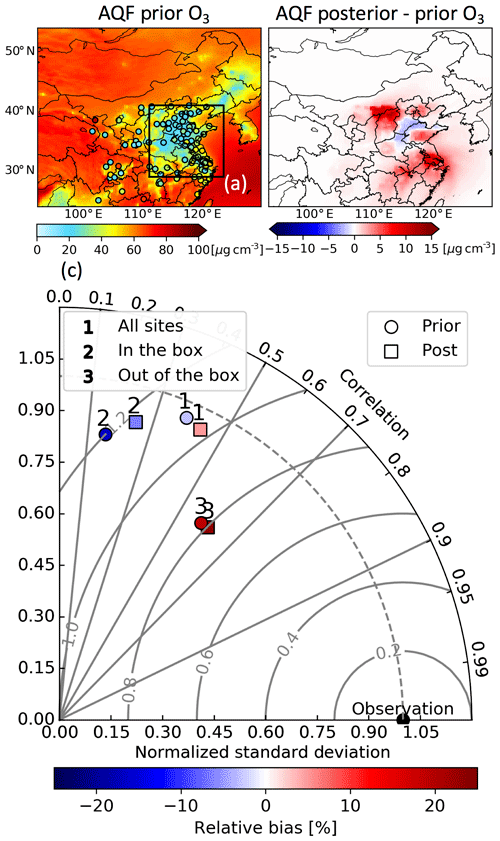Figure 14Evaluation of GEOS-Chem surface O3 forecasts with in situ observations for November 2013. Panel (a) shows AQF-PRI O3 forecasts with in situ observations overlapped. Panel (b) shows the difference between and AQF-MIX-DE-POS and AQF-PRI O3 forecasts. Panel (c) shows the Taylor diagram of evaluations of surface O3 forecasts in (a) and (b) with in situ observations. Circles and squares represent the AQF-PRI and AQF-MIX-DE-POS forecasts, respectively. Labels 1, 2, and 3 represent that all sites, only sites that are within the black box in (a), and only sites that are outside of the black box in (a) are used for evaluations.

Download

In addition to the improvement of SO2 and NO2, AQF-MIX-DE-POS enhances AQF-PRI in the forecast of surface O3 concentrations (Fig. 14). If all O3 in situ observations in the research domain are used for evaluation, a spatial distribution improvement is shown with NCRMSE decreasing from 1.08 for AQF-PRI to 1.05 for AQF-MIX-DE-POS, but NMB changes from −3.1 % to 5.0 % (Fig. 14c). Indeed, whether bias becomes smaller or larger depends on the region. In the North China Plain and eastern China where NOx emissions (or NO2 surface concentrations) are large (the black box in Fig. 14a), forecasts of surface O3 concentration are much lower than in other regions; and the NMB is −16.7 % for AQF-PRI and −6.3 % for AQF-MIX-DE-POS, with NCRMSE decreasing from 1.20 to 1.16 (Fig. 14c). In this relatively NOx-rich region, the increase in O3 concentration in AQF-MIX-DE-POS is caused by the decrease in NO2 concentrations; the change in SO2 concentrations has negligible impacts on O3 concentrations (Fig. S5). This implies that if volatile organic compound (VOC) concentrations remain constant, emission control of NOx emissions will exacerbate O3 pollution. For the region that is outside of the black box, although NCRMSE decreases from 0.82 for AQF-PRI to 0.80 for AQF-MIX-DE-POS, NMB increases from 19.0 % to 23.3 % (Fig. 14c).

5 Discussion and conclusions

The posterior SO2 and NOx emissions at $\mathrm{2}{}^{\circ }×\mathrm{2.5}{}^{\circ }$ resolution constrained by OMPS SO2 and NO2 retrievals through the GEOS-Chem adjoint model (Wang et al., 2020) are expected to improve simulations and forecasts of SO2 and NO2 pollution, but model simulation at such a coarse resolution fails to capture hot spots over cities due to the coarse-grid smearing or CGS effect, which prompts the study and development of downscaling techniques. Here, we introduce several downscaling approaches to obtaining surface SO2 and NO2 concentrations at finer resolution, which are further validated with in situ observations. All these methods are demonstrated through simulations of SO2 and NO2 for October 2013 and forecasts of SO2, NO2, and O3 for November 2013 over China.

GEOS-Chem $\mathrm{2}{}^{\circ }×\mathrm{2.5}{}^{\circ }$ simulations of surface SO2 and NO2 over China in October 2013 using the prior MIX 2010 emissions and the posterior emissions show negative bias due to the coarse-grid smearing (CGS) effect. The coarse-resolution simulations are downscaled to $\mathrm{0.25}{}^{\circ }×\mathrm{0.3125}{}^{\circ }$ resolution according to the distributions of $\mathrm{0.25}{}^{\circ }×\mathrm{0.3125}{}^{\circ }$ simulations based on the prior MIX 2010 emissions (MIX-DDC approach). When comparing with in situ surface observations, the MIX-DDC posterior SO2 and NO2 simulations show the normalized centered root mean squared error (NCRMSE) is 63.7 % and 30.2 %, respectively, lower than the MIX-DDC prior simulations. Compared with the $\mathrm{2}{}^{\circ }×\mathrm{2.5}{}^{\circ }$ simulations, the downscaled $\mathrm{0.25}{}^{\circ }×\mathrm{0.3125}{}^{\circ }$ simulations alleviate the CGS effect but do not avoid it completely. To further decrease the CGS effect for NO2, we further downscale the surface NO2 simulations from $\mathrm{2}{}^{\circ }×\mathrm{2.5}{}^{\circ }$ to $\mathrm{0.05}{}^{\circ }×\mathrm{0.05}{}^{\circ }$ according to VIIRS nighttime light observations, which are strongly related with TROPOMI NO2 tropospheric VCDs (NL-DC approach). The NL-DC NO2 posterior simulation is better than the NL-DC prior simulation when compared with in situ observations with NCRMSE decreasing from 1.34 to 1.10, and the MB decreases from 18.30 to 10.29 µg m−3. In terms of evaluating the downscaled SO2 and NO2 simulations simultaneously, using posterior SO2 and NOx emission inventories from joint assimilation is better than only using one (SO2 or NOx) emission inventory from separate assimilation, and it is similar to using posterior SO2 and NOx emission inventories from separate assimilation.

Instead of using prior fine-resolution simulations to downscale posterior coarse-resolution surface SO2 and NO2 concentrations, another approach is downscaling posterior emissions for $\mathrm{0.25}{}^{\circ }×\mathrm{0.3125}{}^{\circ }$ simulations. We downscale the posterior $\mathrm{2}{}^{\circ }×\mathrm{2.5}{}^{\circ }$ SO2 emissions according to the distributions of fine-resolution prior MIX SO2 emissions (MIX-DE). In the $\mathrm{0.25}{}^{\circ }×\mathrm{0.3125}{}^{\circ }$ simulations, posterior surface SO2 is in better agreement with in situ observations than the prior. Not only are the fine-resolution prior MIX NOx emissions used to downscale posterior $\mathrm{2}{}^{\circ }×\mathrm{2.5}{}^{\circ }$ NOx emissions, we also use VIIRS nighttime light observations as proxies to downscale posterior $\mathrm{2}{}^{\circ }×\mathrm{2.5}{}^{\circ }$ NOx emissions (NL-DE approach). All these emissions are used to simulate surface NO2 concentrations, which are validated with in situ observations. The simulations using MIX-DE and NL-DE posterior NOx emissions show smaller root mean square error and larger linear correlation than the prior simulation. The NO2 simulation using MIX-DE emissions shows better results than that using NL-DE emissions, which may be owing to all NOx emissions being treated as area sources in the NL-DE approach while the MIX-DE approach has point source information, if we assume that sectoral ratios do not change between prior and posterior emissions. We also notice that using the prior fine-resolution simulations to downscale the posterior coarse-resolution surface SO2 and NO2 concentrations is slightly better than simulations using the downscaled posterior emissions.

To study the feasibility of improving surface SO2, NO2, and O3 predictions, posterior emission inventories of the current month are scaled to the next month according to the monthly variations of the prior MIX emission inventory and are subsequently applied to forecasts of the next month. Here we integrate MIX-DE posterior SO2 and NOx emission inventories for October 2013 and the monthly scale factors derived from the prior MIX emission inventory to obtain posterior SO2 and NOx emission inventories for November 2013. These are further used to forecast surface SO2, NO2, and O3 concentrations at $\mathrm{0.25}{}^{\circ }×\mathrm{0.3125}{}^{\circ }$ for November 2013, and the results are better than using prior emissions when evaluated with in situ observations, although the CGS effect is not completely avoided at this spatial resolution for SO2 and NO2. The forecasts of surface NO2 concentrations at $\mathrm{0.05}{}^{\circ }×\mathrm{0.05}{}^{\circ }$ resolutions through NL-DC can eliminate the CGS effect, and the posterior forecast is also in better agreement with in situ observations than the prior forecast.

Data availability

In situ surface observations are available at https://arroma.uiowa.edu/all_datasets.php (last access: 29 May 2020, Wang, 2018) . VIIRS nighttime light data are available at https://ngdc.noaa.gov/eog/viirs/download_dnb_composites.html (last access: 29 May 2020, Elvidge et al., 2017b) . TROPOMI NO2 data are available at https://disc.gsfc.nasa.gov/datasets/S5P_L2__NO2____1/summary (last access: 29 May 2020, KNMI, 2018). The MIX emission inventory is available at http://geoschemdata.computecanada.ca/ExtData/HEMCO/MIX/v2015-03/ (last access: 29 May 2020, Li et al., 2017b). The downscaled SO2 and NOx emission inventories are available at https://arroma.uiowa.edu/all_datasets.php (last access: 29 May 2020, Wang et al., 2019).

Supplement

The supplement related to this article is available online at: https://doi.org/10.5194/acp-20-6651-2020-supplement.

Author contributions

YW, JW, and DKH designed the research; YW conducted the research; YW and JW wrote the paper; DKH contributed to writing; CG participated in the GEOS-Chem simulation; MZ prepared VIIRS nighttime light data; and WW prepared in situ observations.

Competing interests

The authors declare that they have no conflict of interest.

Acknowledgements

This research is supported by the National Aeronautics and Space Administration (NASA) through the Aura program managed by Kenneth W. Jucks, the ACMAP program (grant number NNX17AF77G and grant 80NSSC19K0950) managed by Richard Eckman, and the TEMPO project as part of NASA's Earth Venture program (grant number SV7-87011 subcontracted from the Harvard–Smithsonian Observatory to The University of Iowa). We acknowledge the computational support from the High-Performance Computing group at The University of Iowa.

Financial support

This research has been supported by NASA (grant nos. 80NSSC19K0950, NNX17AF77G, and SV7-87011).

Review statement

This paper was edited by Leiming Zhang and reviewed by two anonymous referees.

References

Bechle, M. J., Millet, D. B., and Marshall, J. D.: Remote sensing of exposure to NO2: Satellite versus ground-based measurement in a large urban area, Atmos. Environ., 69, 345–353, https://doi.org/10.1016/j.atmosenv.2012.11.046, 2013.

Bennett, M. M. and Smith, L. C.: Advances in using multitemporal night-time lights satellite imagery to detect, estimate, and monitor socioeconomic dynamics, Remote Sens. Environ., 192, 176–197, https://doi.org/10.1016/j.rse.2017.01.005, 2017.

Ding, J., van der A, R. J., Mijling, B., Levelt, P. F., and Hao, N.: NOx emission estimates during the 2014 Youth Olympic Games in Nanjing, Atmos. Chem. Phys., 15, 9399–9412, https://doi.org/10.5194/acp-15-9399-2015, 2015.

Elvidge, C. D., Baugh, K., Zhizhin, M., Hsu, F. C., and Ghosh, T.: VIIRS night-time lights, Int. J. Remote Sens., 38, 5860–5879, https://doi.org/10.1080/01431161.2017.1342050, 2017a.

Elvidge, C. D., Baugh, K., Zhizhin, M., Hsu, F. C., and Ghosh, T.: VIIRS night-time lights, available: https://ngdc.noaa.gov/eog/viirs/download_dnb_composites.html (last access: 29 May 2020), 2017b.

Fioletov, V. E., McLinden, C. A., Krotkov, N., Li, C., Joiner, J., Theys, N., Carn, S., and Moran, M. D.: A global catalogue of large SO2 sources and emissions derived from the Ozone Monitoring Instrument, Atmos. Chem. Phys., 16, 11497–11519, https://doi.org/10.5194/acp-16-11497-2016, 2016.

Gao, M., Carmichael, G. R., Wang, Y., Ji, D., Liu, Z., and Wang, Z.: Improving simulations of sulfate aerosols during winter haze over Northern China: the impacts of heterogeneous oxidation by NO2, Front. Environ. Sci. Eng., 10, 16, https://doi.org/10.1007/s11783-016-0878-2, 2016.

Ge, C., Wang, J., Reid, J. S., Posselt, D. J., Xian, P., and Hyer, E.: Mesoscale modeling of smoke transport from equatorial Southeast Asian Maritime Continent to the Philippines: First comparison of ensemble analysis with in situ observations, J. Geophys. Res., 122, 5380–5398, https://doi.org/10.1002/2016JD026241, 2017.

Geng, G., Zhang, Q., Martin, R. V., Lin, J., Huo, H., Zheng, B., Wang, S., and He, K.: Impact of spatial proxies on the representation of bottom-up emission inventories: A satellite-based analysis, Atmos. Chem. Phys., 17, 4131–4145, https://doi.org/10.5194/acp-17-4131-2017, 2017.

Ghozikali, G. M., Heibati, B., Naddafi, K., Kloog, I., Oliveri Conti, G., Polosa, R., and Ferrante, M.: Evaluation of Chronic Obstructive Pulmonary Disease (COPD) attributed to atmospheric O3, NO2, and SO2 using Air Q Model (2011–2012 year), Environ. Res., 144, 99–105, https://doi.org/10.1016/j.envres.2015.10.030, 2016.

Goldberg, D. L., Lamsal, L. N., Loughner, C. P., Swartz, W. H., Lu, Z., and Streets, D. G.: A high-resolution and observationally constrained OMI NO2 satellite retrieval, Atmos. Chem. Phys., 17, 11403–11421, https://doi.org/10.5194/acp-17-11403-2017, 2017.

Goldberg, D. L., Saide, P. E., Lamsal, L. N., de Foy, B., Lu, Z., Woo, J.-H., Kim, Y., Kim, J., Gao, M., Carmichael, G., and Streets, D. G.: A top-down assessment using OMI NO2 suggests an underestimate in the NOx emissions inventory in Seoul, South Korea, during KORUS-AQ, Atmos. Chem. Phys., 19, 1801–1818, https://doi.org/10.5194/acp-19-1801-2019, 2019.

Griffin, D., Zhao, X., McLinden, C. A., Boersma, F., Bourassa, A., Dammers, E., Degenstein, D., Eskes, H., Fehr, L., Fioletov, V., Hayden, K., Kharol, S. K., Li, S.-M., Makar, P., Martin, R. V., Mihele, C., Mittermeier, R. L., Krotkov, N., Sneep, M., Lamsal, L. N., Linden, M. t., Geffen, J. v., Veefkind, P., and Wolde, M.: High-Resolution Mapping of Nitrogen Dioxide With TROPOMI: First Results and Validation Over the Canadian Oil Sands, Geophys. Res. Lett., 46, 1049–1060, https://doi.org/10.1029/2018GL081095, 2019.

Henze, D. K., Hakami, A., and Seinfeld, J. H.: Development of the adjoint of GEOS-Chem, Atmos. Chem. Phys., 7, 2413–2433, https://doi.org/10.5194/acp-7-2413-2007, 2007.

Hong, C., Zhang, Q., Zhang, Y., Tang, Y., Tong, D., and He, K.: Multi-year downscaling application of two-way coupled WRF v3.4 and CMAQ v5.0.2 over east Asia for regional climate and air quality modeling: model evaluation and aerosol direct effects, Geosci. Model Dev., 10, 2447–2470, https://doi.org/10.5194/gmd-10-2447-2017, 2017.

Huang, X., Song, Y., Li, M., Li, J., Huo, Q., Cai, X., Zhu, T., Hu, M., and Zhang, H.: A high-resolution ammonia emission inventory in China, Global Biogeochem. Cy., 26, GB1030, https://doi.org/10.1029/2011GB004161, 2012.

Janssens-Maenhout, G., Crippa, M., Guizzardi, D., Dentener, F., Muntean, M., Pouliot, G., Keating, T., Zhang, Q., Kurokawa, J., Wankmüller, R., Denier van der Gon, H., Kuenen, J. J. P., Klimont, Z., Frost, G., Darras, S., Koffi, B., and Li, M.: HTAP_v2.2: a mosaic of regional and global emission grid maps for 2008 and 2010 to study hemispheric transport of air pollution, Atmos. Chem. Phys., 15, 11411–11432, https://doi.org/10.5194/acp-15-11411-2015, 2015.

Keller, C. A., Long, M. S., Yantosca, R. M., Da Silva, A. M., Pawson, S., and Jacob, D. J.: HEMCO v1.0: a versatile, ESMF-compliant component for calculating emissions in atmospheric models, Geosci. Model Dev., 7, 1409–1417, https://doi.org/10.5194/gmd-7-1409-2014, 2014.

Kharol, S. K., Martin, R. V., Philip, S., Boys, B., Lamsal, L. N., Jerrett, M., Brauer, M., Crouse, D. L., McLinden, C., and Burnett, R. T.: Assessment of the magnitude and recent trends in satellite-derived ground-level nitrogen dioxide over North America, Atmos. Environ., 118, 236–245, https://doi.org/10.1016/j.atmosenv.2015.08.011, 2015.

Kharol, S. K., McLinden, C. A., Sioris, C. E., Shephard, M. W., Fioletov, V., van Donkelaar, A., Philip, S., and Martin, R. V.: OMI satellite observations of decadal changes in ground-level sulfur dioxide over North America, Atmos. Chem. Phys., 17, 5921–5929, https://doi.org/10.5194/acp-17-5921-2017, 2017.

Kim, H., Lee, S.-M., Chai, T., Ngan, F., Pan, L., and Lee, P.: A Conservative Downscaling of Satellite-Detected Chemical Compositions: NO2 Column Densities of OMI, GOME-2, and CMAQ, Remote Sensing, 10, 1001, https://doi.org/10.3390/rs10071001, 2018.

Kim, H. C., Lee, P., Judd, L., Pan, L., and Lefer, B.: OMI NO2 column densities over North American urban cities: the effect of satellite footprint resolution, Geosci. Model Dev., 9, 1111–1123, https://doi.org/10.5194/gmd-9-1111-2016, 2016.

Kong, H., Lin, J., Zhang, R., Liu, M., Weng, H., Ni, R., Chen, L., Wang, J., Yan, Y., and Zhang, Q.: High-resolution ($\mathrm{0.05}{}^{\circ }×\mathrm{ }\mathrm{ }\mathrm{0.05}{}^{\circ }$) NOx emissions in the Yangtze River Delta inferred from OMI, Atmos. Chem. Phys., 19, 12835–12856, https://doi.org/10.5194/acp-19-12835-2019, 2019.

Koninklijk Nederlands Meteorologisch Instituut (KNMI): Sentinel-5P TROPOMI Tropospheric NO2 1-Orbit L2 7 km × 3.5 km, Greenbelt, MD, USA, Goddard Earth Sciences Data and Information Services Center (GES DISC), available at: https://disc.gsfc.nasa.gov/datasets/S5P_L2__NO2____1/summary (last access: 29 May 2020), 2018.

Koukouli, M. E., Theys, N., Ding, J., Zyrichidou, I., Mijling, B., Balis, D., and van der A, R. J.: Updated SO2 emission estimates over China using OMI/Aura observations, Atmos. Meas. Tech., 11, 1817–1832, https://doi.org/10.5194/amt-11-1817-2018, 2018.

Kurokawa, J., Ohara, T., Morikawa, T., Hanayama, S., Janssens-Maenhout, G., Fukui, T., Kawashima, K., and Akimoto, H.: Emissions of air pollutants and greenhouse gases over Asian regions during 2000–2008: Regional Emission inventory in ASia (REAS) version 2, Atmos. Chem. Phys., 13, 11019–11058, https://doi.org/10.5194/acp-13-11019-2013, 2013.

Kurokawa, J.-i., Yumimoto, K., Uno, I., and Ohara, T.: Adjoint inverse modeling of NOx emissions over eastern China using satellite observations of NO2 vertical column densities, Atmos. Environ., 43, 1878–1887, https://doi.org/10.1016/j.atmosenv.2008.12.030, 2009.

Lamsal, L. N., Martin, R. V., van Donkelaar, A., Steinbacher, M., Celarier, E. A., Bucsela, E., Dunlea, E. J., and Pinto, J. P.: Ground-level nitrogen dioxide concentrations inferred from the satellite-borne Ozone Monitoring Instrument, J. Geophys. Res., 113, D16308, https://doi.org/10.1029/2007JD009235, 2008.

Lamsal, L. N., Martin, R. V., Padmanabhan, A., van Donkelaar, A., Zhang, Q., Sioris, C. E., Chance, K., Kurosu, T. P., and Newchurch, M. J.: Application of satellite observations for timely updates to global anthropogenic NOx emission inventories, Geophys. Res. Lett., 38, L05810, https://doi.org/10.1029/2010GL046476, 2011.

Lee, C., Martin, R. V., van Donkelaar, A., Lee, H., Dickerson, R. R., Hains, J. C., Krotkov, N., Richter, A., Vinnikov, K., and Schwab, J. J.: SO2 emissions and lifetimes: Estimates from inverse modeling using in situ and global, space-based (SCIAMACHY and OMI) observations, J. Geophys. Res., 116, D06304, https://doi.org/10.1029/2010JD014758, 2011.

Levin, N. and Zhang, Q.: A global analysis of factors controlling VIIRS nighttime light levels from densely populated areas, Remote Sens. Environ., 190, 366–382, https://doi.org/10.1016/j.rse.2017.01.006, 2017.

Li, M., Zhang, Q., Kurokawa, J.-I., Woo, J.-H., He, K., Lu, Z., Ohara, T., Song, Y., Streets, D. G., Carmichael, G. R., Cheng, Y., Hong, C., Huo, H., Jiang, X., Kang, S., Liu, F., Su, H., and Zheng, B.: MIX: a mosaic Asian anthropogenic emission inventory under the international collaboration framework of the MICS-Asia and HTAP, Atmos. Chem. Phys., 17, 935–963, https://doi.org/10.5194/acp-17-935-2017, 2017a.

Li, M., Zhang, Q., Kurokawa, J. I., Woo, J. H., He, K., Lu, Z., Ohara, T.,Song, Y., Streets, D. G., Carmichael, G. R., Cheng, Y., Hong, C., Huo, H., Jiang, X., Kang, S., Liu, F., Su, H., and Zheng, B.: The MIX emission inventory for GEOS-Chem simulation, available at: http://geoschemdata.computecanada.ca/ExtData/HEMCO/MIX/v2015-03/ (last access: 29 May 2020), 2017b.

Lin, J.-T., McElroy, M. B., and Boersma, K. F.: Constraint of anthropogenic NOx emissions in China from different sectors: a new methodology using multiple satellite retrievals, Atmos. Chem. Phys., 10, 63–78, https://doi.org/10.5194/acp-10-63-2010, 2010.

Liu, F., Choi, S., Li, C., Fioletov, V. E., McLinden, C. A., Joiner, J., Krotkov, N. A., Bian, H., Janssens-Maenhout, G., Darmenov, A. S., and da Silva, A. M.: A new global anthropogenic SO2 emission inventory for the last decade: a mosaic of satellite-derived and bottom-up emissions, Atmos. Chem. Phys., 18, 16571–16586, https://doi.org/10.5194/acp-18-16571-2018, 2018.

Liu, F., Zhang, Q., Tong, D., Zheng, B., Li, M., Huo, H., and He, K. B.: High-resolution inventory of technologies, activities, and emissions of coal-fired power plants in China from 1990 to 2010, Atmos. Chem. Phys., 15, 13299–13317, https://doi.org/10.5194/acp-15-13299-2015, 2015.

Lu, Z. and Streets, D. G.: Increase in NOx Emissions from Indian Thermal Power Plants during 1996–2010: Unit-Based Inventories and Multisatellite Observations, Environ. Sci. Technol., 46, 7463–7470, https://doi.org/10.1021/es300831w, 2012.

Lu, Z., Zhang, Q., and Streets, D. G.: Sulfur dioxide and primary carbonaceous aerosol emissions in China and India, 1996–2010, Atmos. Chem. Phys., 11, 9839–9864, https://doi.org/10.5194/acp-11-9839-2011, 2011.

Martin, R. V., Jacob, D. J., Chance, K., Kurosu, T. P., Palmer, P. I., and Evans, M. J.: Global inventory of nitrogen oxide emissions constrained by space-based observations of NO2 columns, J. Geophys. Res., 108, 4537, https://doi.org/10.1029/2003JD003453, 2003.

Mijling, B. and van der A, R. J.: Using daily satellite observations to estimate emissions of short-lived air pollutants on a mesoscopic scale, J. Geophys. Res., 117, D17302, https://doi.org/10.1029/2012JD017817, 2012.

Miller, D. S., Straka, W., Mills, P. S., Elvidge, D. C., Lee, F. T., Solbrig, J., Walther, A., Heidinger, K. A., and Weiss, C. S.: Illuminating the Capabilities of the Suomi National Polar-Orbiting Partnership (NPP) Visible Infrared Imaging Radiometer Suite (VIIRS) Day/Night Band, Remote Sensing, 5, 6717–6766, https://doi.org/10.3390/rs5126717, 2013.

Miyazaki, K., Eskes, H. J., Sudo, K., Takigawa, M., van Weele, M., and Boersma, K. F.: Simultaneous assimilation of satellite NO2, O3, CO, and HNO3 data for the analysis of tropospheric chemical composition and emissions, Atmos. Chem. Phys., 12, 9545–9579, https://doi.org/10.5194/acp-12-9545-2012, 2012.

Miyazaki, K., Eskes, H., Sudo, K., Boersma, K. F., Bowman, K., and Kanaya, Y.: Decadal changes in global surface NOx emissions from multi-constituent satellite data assimilation, Atmos. Chem. Phys., 17, 807–837, https://doi.org/10.5194/acp-17-807-2017, 2017.

Ou, J., Liu, X., Li, X., Li, M., and Li, W.: Evaluation of NPP-VIIRS Nighttime Light Data for Mapping Global Fossil Fuel Combustion CO2 Emissions: A Comparison with DMSP-OLS Nighttime Light Data, PLOS ONE, 10, e0138310, https://doi.org/10.1371/journal.pone.0138310, 2015.

Palmer, P. I., Jacob, D. J., Fiore, A. M., Martin, R. V., Chance, K., and Kurosu, T. P.: Mapping isoprene emissions over North America using formaldehyde column observations from space, J. Geophys. Res., 108, 4180, https://doi.org/10.1029/2002JD002153, 2003.

Qu, Z., Henze, D. K., Capps, S. L., Wang, Y., Xu, X., Wang, J., and Keller, M.: Monthly top-down NOx emissions for China (2005–2012): A hybrid inversion method and trend analysis, J. Geophys. Res., 122, 4600–4625, https://doi.org/10.1002/2016JD025852, 2017.

Qu, Z., Henze, D. K., Li, C., Theys, N., Wang, Y., Wang, J., Wang, W., Han, J., Shim, C., Dickerson, R. R., and Ren, X.: SO2 emission estimates using OMI SO2 retrievals for 2005–2017, J. Geophys. Res., 124, 8336–8359, https://doi.org/10.1029/2019JD030243, 2019a.

Qu, Z., Henze, D. K., Theys, N., Wang, J., and Wang, W.: Hybrid mass balance/4D-Var joint inversion of NOx and SO2 emissions in East Asia, J. Geophys. Res., 124, 8203–8224, https://doi.org/10.1029/2018JD030240, 2019b.

Steinbacher, M., Zellweger, C., Schwarzenbach, B., Bugmann, S., Buchmann, B., Ordóñez, C., Prevot, A. S. H., and Hueglin, C.: Nitrogen oxide measurements at rural sites in Switzerland: Bias of conventional measurement techniques, J. Geophys. Res., 112, D11307, https://doi.org/10.1029/2006JD007971, 2007.

Streets, D. G., Bond, T. C., Carmichael, G. R., Fernandes, S. D., Fu, Q., He, D., Klimont, Z., Nelson, S. M., Tsai, N. Y., Wang, M. Q., Woo, J. H., and Yarber, K. F.: An inventory of gaseous and primary aerosol emissions in Asia in the year 2000, J. Geophys. Res., 108, 8809, https://doi.org/10.1029/2002JD003093, 2003.

Turner, A. J., Henze, D. K., Martin, R. V., and Hakami, A.: The spatial extent of source influences on modeled column concentrations of short-lived species, Geophys. Res. Lett., 39, L12806, https://doi.org/10.1029/2012GL051832, 2012.

Wang, J., Xu, X., Henze, D. K., Zeng, J., Ji, Q., Tsay, S.-C., and Huang, J.: Top-down estimate of dust emissions through integration of MODIS and MISR aerosol retrievals with the GEOS-Chem adjoint model, Geophys. Res. Lett., 39, L08802, https://doi.org/10.1029/2012GL051136, 2012.

Wang, W.: In situ surface SO2, NO2, and O3 observations over China, available at: https://arroma.uiowa.edu/all_datasets.php (last access: 29 May 2020), 2018.

Wang, Y., Wang, J., Xu, X., Henze, D. K., Wang, Y., and Qu, Z.: A new approach for monthly updates of anthropogenic sulfur dioxide emissions from space: Application to China and implications for air quality forecasts, Geophys. Res. Lett., 43, 9931–9938, https://doi.org/10.1002/2016GL070204, 2016a.

Wang, J., Aegerter, C., Xu, X., and Szykman, J. J.: Potential application of VIIRS Day/Night Band for monitoring nighttime surface PM2.5 air quality from space, Atmos. Environ., 124, 55–63, https://doi.org/10.1016/j.atmosenv.2015.11.013, 2016b.

Wang, Y., Wang, J., Zhou, M., Henze, D. K., Ge, C., and Wang, W.: The downscaled SO2 and NOx emission inventories, available: https://arroma.uiowa.edu/all_datasets.php (last access: 29 May 2020), 2019.

Wang, Y., Wang, J., Xu, X., Henze, D. K., Qu, Z., and Yang, K.: Inverse modeling of SO2 and NOx emissions over China using multisensor satellite data – Part 1: Formulation and sensitivity analysis, Atmos. Chem. Phys., 20, 6631–6650, https://doi.org/10.5194/acp-20-6631-2020, 2020.

Xing, J., Mathur, R., Pleim, J., Hogrefe, C., Gan, C.-M., Wong, D. C., Wei, C., Gilliam, R., and Pouliot, G.: Observations and modeling of air quality trends over 1990–2010 across the Northern Hemisphere: China, the United States and Europe, Atmos. Chem. Phys., 15, 2723–2747, https://doi.org/10.5194/acp-15-2723-2015, 2015.

Xu, X., Wang, J., Henze, D. K., Qu, W., and Kopacz, M.: Constraints on aerosol sources using GEOS-Chem adjoint and MODIS radiances, and evaluation with multisensor (OMI, MISR) data, J. Geophys. Res., 118, 6396–6413, https://doi.org/10.1002/jgrd.50515, 2013.

Yahya, K., Wang, K., Campbell, P., Glotfelty, T., He, J., and Zhang, Y.: Decadal evaluation of regional climate, air quality, and their interactions over the continental US and their interactions using WRF/Chem version 3.6.1, Geosci. Model Dev., 9, 671–695, https://doi.org/10.5194/gmd-9-671-2016, 2016.

Yahya, K., Wang, K., Campbell, P., Chen, Y., Glotfelty, T., He, J., Pirhalla, M., and Zhang, Y.: Decadal application of WRF/Chem for regional air quality and climate modeling over the U.S. under the representative concentration pathways scenarios. Part 1: Model evaluation and impact of downscaling, Atmos. Environ., 152, 562–583, https://doi.org/10.1016/j.atmosenv.2016.12.029, 2017.

Zhang, Y.-L. and Cao, F.: Fine particulate matter (PM2.5) in China at a city level, Sci. Rep., 5, 14884, https://doi.org/10.1038/srep14884, 2015.

Zheng, B., Zhang, Q., Tong, D., Chen, C., Hong, C., Li, M., Geng, G., Lei, Y., Huo, H., and He, K.: Resolution dependence of uncertainties in gridded emission inventories: a case study in Hebei, China, Atmos. Chem. Phys., 17, 921–933, https://doi.org/10.5194/acp-17-921-2017, 2017.

Zheng, B., Tong, D., Li, M., Liu, F., Hong, C., Geng, G., Li, H., Li, X., Peng, L., Qi, J., Yan, L., Zhang, Y., Zhao, H., Zheng, Y., He, K., and Zhang, Q.: Trends in China's anthropogenic emissions since 2010 as the consequence of clean air actions, Atmos. Chem. Phys., 18, 14095–14111, https://doi.org/10.5194/acp-18-14095-2018, 2018.